# Implications of Interactions in Treatment Comparisons

RCT
drug-evaluation
generalizability
medicine
observational
personalized-medicine
prediction
subgroup
2020
This article explains how the generalizability of randomized trial findings depends primarily on whether and how patient characteristics modify (interact with) the treatment effect. For an observational study this will be related to overlap in the propensity to receive treatment.
Author
Affiliation

Vanderbilt University
School of Medicine
Department of Biostatistics

Published

March 3, 2020

Transportability of treatment effect estimates depends on the nature of interactions. In the absence of interactions, an effect estimated on a highly selected sample will apply to a much different population. In an observational study, the corresponding condition is that there need be no overlap in the baseline distribution of non-interacting factors. When there is an interaction, one can live with only a small to moderate amount of overlap in characteristics (between randomized vs. population target or between characteristics of treated vs. non-treated patients in an observational study) if the interaction is of a simple form. With more overlap, interactions can be complex (if modeled) and results from analysis of the sample will allow estimation of treatment effect in a different population. In the absence of significant overlap, confidence bands allowing for interaction properly inform the researcher about uncertainties in treatment effects.

Randomized clinical trials do not require representative patients; they require representative treatment effects. Generalizability of randomized trial findings for relative efficacy comes from one of three things:

1. true absence of interactions,
2. interacting factors have a similar distribution in the RCT as in the target population, or
3. the RCT sample has enough representation of the distribution of interacting factors to allow them to be modeled and used to estimate treatment effects in target patients, and the researcher knows to include these interactions in her model.

Note: For shaded boxes marked with ⮩ click on the box to view the associated text.

# Background

It is a commonly held belief that clinical trials, to provide treatment effects that are generalizable to a population, must use a sample that reflects that population’s characteristics. The confusion stems from the fact that if one were interested in estimating an average outcome for patients given treatment A, one would need a random sample from the target population. But clinical trials are not designed to estimate absolutes; they are designed to estimate differences as discussed further here. These differences, when measured on a scale for which treatment differences are allowed mathematically to be constant (e.g., difference in means, odds ratios, hazard ratios), show remarkable constancy as judged by a large number of published forest plots. What would make a treatment estimate (relative efficacy) not be transportable to another population? A requirement for non-generalizability is the existence of interactions with treatment such that the interacting factors have a distribution in the sample that is much different from the distribution in the population.

A related problem is the issue of overlap in observational studies. Researchers are taught that non-overlap makes observational treatment comparisons impossible. This is only true when the characteristic whose distributions don’t overlap between treatment groups interacts with treatment. The purpose of this article is to explore interactions in these contexts.

As a side note, if there is an interaction between treatment and a covariate, standard propensity score analysis will completely miss it.

For the following I assume that there is at most one variable (patient age) interacting with treatment effect. The data generating and analysis models are logistic models containing only age and treatment. I also assume that the goal is to assess efficacy isolated from net clinical benefit, e.g., we are not taking side effects into account.

Situations addressed below come from the following 2 × 2 × 2 × 3 combinations:

• randomized vs. observational study
• true data generating process has no interaction with age vs. has a linear interaction
• overlap in ages between RCT sample and target population (or between treatments in an observational study) is completely absent vs. partial overlap in the age distribution
• fitted model is linear with no interaction, has a linear interaction, or has a spline function of age interacting with treatment

I’ll explore what happens when interaction should be included in a model but is omitted, when an interaction is not needed but is included in the model, and when the interaction is simple but is allowed to be complex.

# Generalizability of Randomized Clinical Trials

When the outcome generating process in a randomized clinical trial (RCT) is such that treatment does not interact with any baseline patient characteristic over the range of characteristics seen in the union of the RCT and the population, the estimate of relative efficacy (e.g., odds ratio) of treatment applies to every patient in the population. In addition to computing patient-specific odds ratios (ORs), one can easily compute patient-specific absolute risk reductions from treatment as shown here. If one allows for needless interaction terms, it is possible to get poor extrapolation of treatment benefit in unrepresented patient groups, unless the estimated interaction effects are very small (not guaranteed until the sample size is very large).

## True Model Has No Treatment Interactions

### Estimation of Treatment Effect in Population With No Overlap

As an example, consider a simulated study of about 2500 patients with a binary outcome where the model contains only age and treatment, the age effect is linear, and there is no interaction. The following R code simulates the data from a hypothetical population, fits a binary logistic model, and displays estimated log odds of outcome vs. age and treatment (left panel) and the estimated age-specific treatment effect (right panel), along with the true population values for both panels over the whole range of age. The RCT excluded those with age < 50 but the target population is exactly those patients. Tick marks on fitted curves display treatment-specific raw data spike histograms.

The original age range is 13.3 - 88.1 in 5000 subjects, and the clinical trial includes 2461 of the subjects.

Age distributions in the target population as compared to the RCT sample are shown below.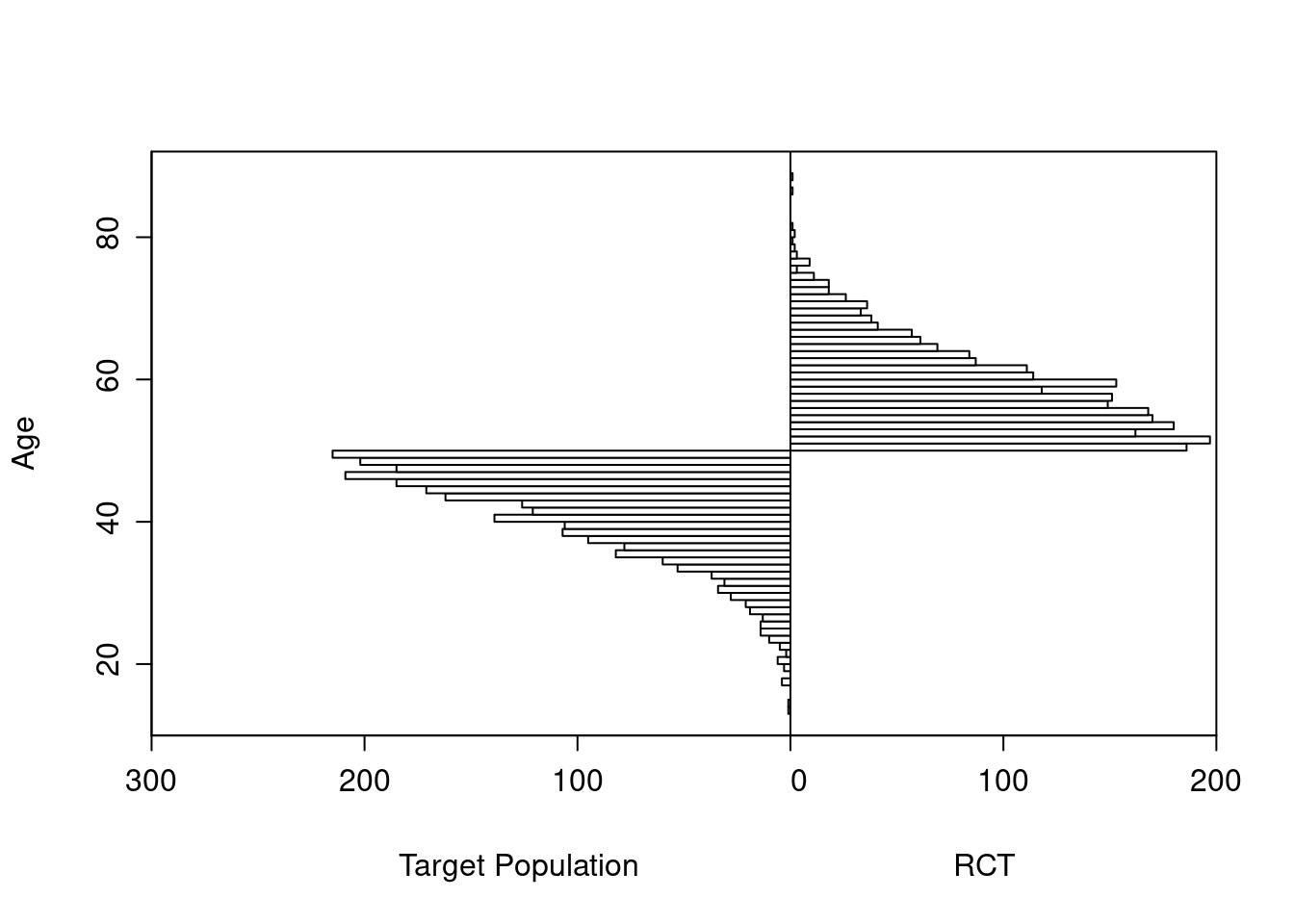Logistic Regression Model

``` lrm(formula = y ~ tx + age, data = d)
```

Frequencies of Missing Values Due to Each Variable

```    y   tx  age
2539    0    0
```
Model Likelihood
Ratio Test
Discrimination
Indexes
Rank Discrim.
Indexes
Obs 2461 LR χ2 211.90 R2 0.117 C 0.679
0 1735 d.f. 2 R22,2461 0.082 Dxy 0.357
1 726 Pr(>χ2) <0.0001 R22,1535.5 0.128 γ 0.357
max |∂log L/∂β| 2×10-7 Brier 0.190 τa 0.149
β S.E. Wald Z Pr(>|Z|)
Intercept  -4.3508  0.4387 -9.92 <0.0001
tx=b  -1.0992  0.0959 -11.46 <0.0001
age   0.0674  0.0074 9.05 <0.0001
 χ2 d.f. P Wald Statistics for `y` tx 131.30 1 <0.0001 age 81.95 1 <0.0001 TOTAL 189.63 2 <0.0001Extrapolation to the younger population is fine even with no younger patients in the RCT. Confidence bands are computed under the assumption of an additive linear age effect.

This is the correct model.

Logistic Regression Model

``` lrm(formula = y ~ tx * age, data = d)
```

Frequencies of Missing Values Due to Each Variable

```    y   tx  age
2539    0    0
```
Model Likelihood
Ratio Test
Discrimination
Indexes
Rank Discrim.
Indexes
Obs 2461 LR χ2 212.02 R2 0.117 C 0.679
0 1735 d.f. 3 R23,2461 0.081 Dxy 0.357
1 726 Pr(>χ2) <0.0001 R23,1535.5 0.127 γ 0.357
max |∂log L/∂β| 5×10-6 Brier 0.190 τa 0.149
β S.E. Wald Z Pr(>|Z|)
Intercept  -4.2193  0.5852 -7.21 <0.0001
tx=b  -1.3998  0.8939 -1.57 0.1174
age   0.0652  0.0100 6.53 <0.0001
tx=b × age   0.0051  0.0150 0.34 0.7351
 χ2 d.f. P Wald Statistics for `y` tx (Factor+Higher Order Factors) 131.11 2 <0.0001 All Interactions 0.11 1 0.7351 age (Factor+Higher Order Factors) 82.19 2 <0.0001 All Interactions 0.11 1 0.7351 tx × age (Factor+Higher Order Factors) 0.11 1 0.7351 TOTAL 188.87 3 <0.0001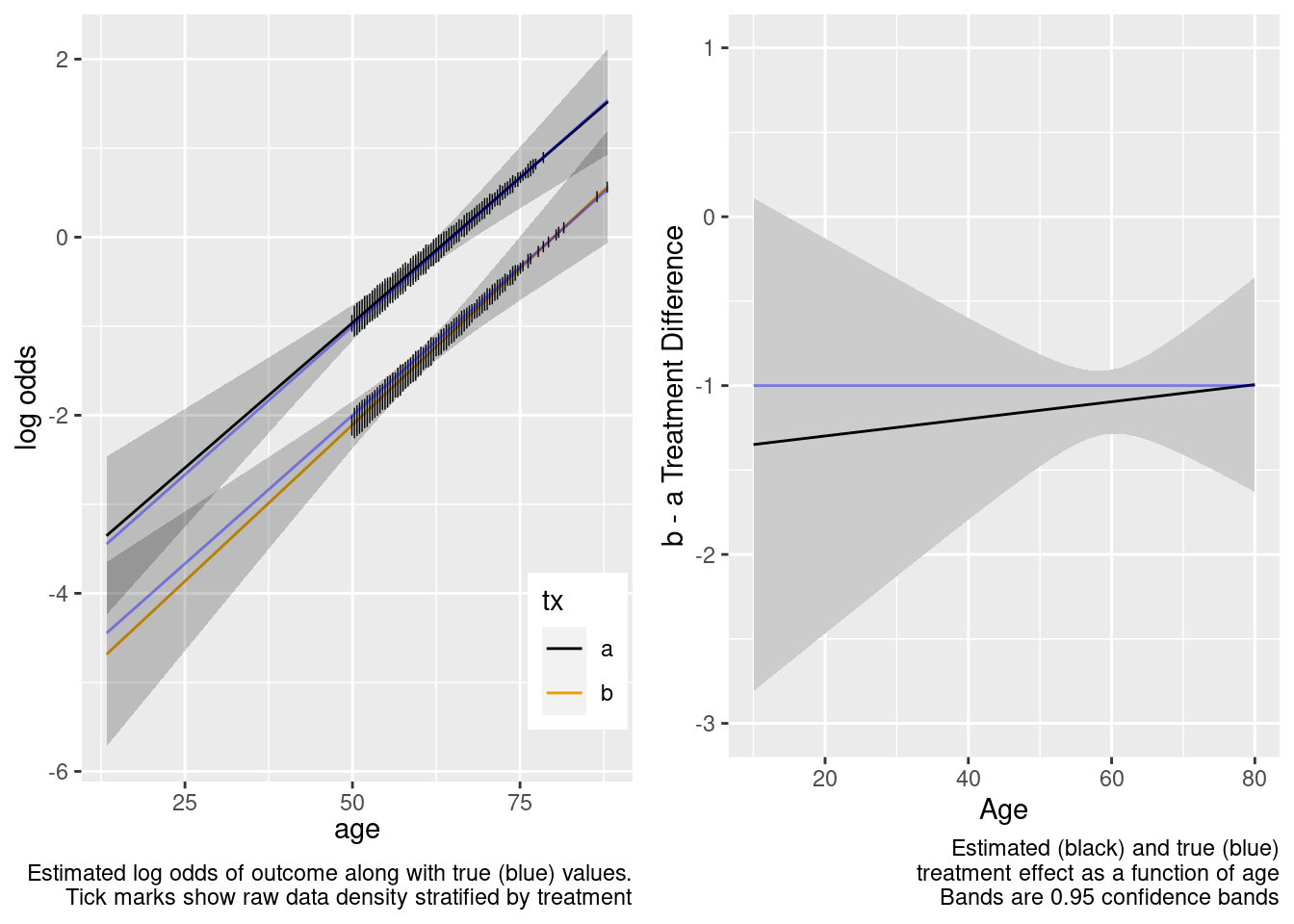Note that the close-to-zero estimated interaction in the trial sample led to an appropriate extrapolation to the target population of age < 50, with the confidence bands getting wider.

Now see what happens when age is unnecessarily allowed to have a nonlinear non-additive effect, by fitting a model that is quadratic in age interacting with treatment.

This model included an unnecessary linear interaction term.

Logistic Regression Model

``` lrm(formula = form, data = d, tol = 1e-12)
```

Frequencies of Missing Values Due to Each Variable

```    y   tx  age
2539    0    0
```
Model Likelihood
Ratio Test
Discrimination
Indexes
Rank Discrim.
Indexes
Obs 2461 LR χ2 214.13 R2 0.119 C 0.679
0 1735 d.f. 7 R27,2461 0.081 Dxy 0.358
1 726 Pr(>χ2) <0.0001 R27,1535.5 0.126 γ 0.358
max |∂log L/∂β| 2×10-5 Brier 0.190 τa 0.149
β S.E. Wald Z Pr(>|Z|)
Intercept  -3.0988  1.2779 -2.42 0.0153
tx=b  -1.3609  2.0910 -0.65 0.5151
age   0.0445  0.0231 1.93 0.0542
age’   0.2124  0.2213 0.96 0.3372
age’’  -0.4384  0.4906 -0.89 0.3716
tx=b × age   0.0043  0.0376 0.11 0.9087
tx=b × age’   0.0067  0.3136 0.02 0.9829
tx=b × age’’  -0.0141  0.6574 -0.02 0.9829
 χ2 d.f. P Wald Statistics for `y` tx (Factor+Higher Order Factors) 131.33 4 <0.0001 All Interactions 0.11 3 0.9910 age (Factor+Higher Order Factors) 85.26 6 <0.0001 All Interactions 0.11 3 0.9910 Nonlinear (Factor+Higher Order Factors) 2.11 4 0.7151 tx × age (Factor+Higher Order Factors) 0.11 3 0.9910 Nonlinear 0.00 2 0.9998 Nonlinear Interaction : f(A,B) vs. AB 0.00 2 0.9998 TOTAL NONLINEAR 2.11 4 0.7151 TOTAL NONLINEAR + INTERACTION 2.23 5 0.8162 TOTAL 190.78 7 <0.0001By allowing not only an unnecessary interaction but also allowing the interaction to be nonlinear, when the predicted values are for a region completely outside the sample data, the extrapolation is still reasonable but the (honest) confidence intervals are much wider. We don’t know much at all about the relative treatment effect with age < 50 when we allowed the two treatments to have differently shaped age effects in the trial data. A Bayesian model that put a skeptical prior on either the nonlinear or the interaction effect would have credible intervals that are not so wide on the left.

This model included unnecessary linear and nonlinear interaction terms.

### RCT Sample Partially Overlaps with Target Population

Instead of having non-overlapping age distributions between the RCT sample and the target population, let’s include screened patients with probabilities that are functions of age as shown in the first graph below. Then again consider the simplest logistic model.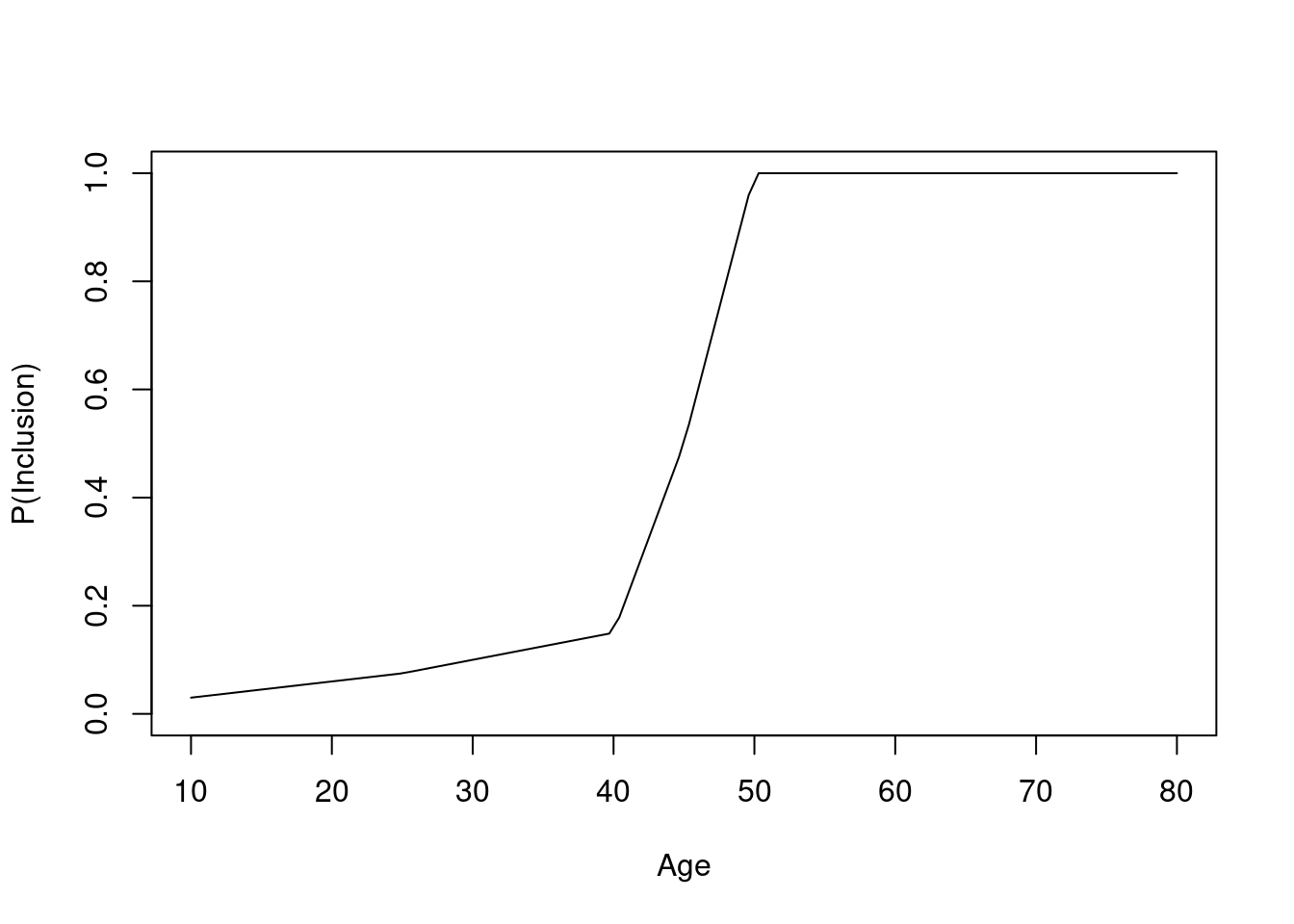The original age range is 13.3 - 88.1 in 5000 subjects, and the clinical trial includes 3556 of the subjects.

Age distributions in the target population as compared to the RCT sample are shown below.Logistic Regression Model

``` lrm(formula = y ~ tx + age, data = d)
```

Frequencies of Missing Values Due to Each Variable

```    y   tx  age
1444    0    0
```
Model Likelihood
Ratio Test
Discrimination
Indexes
Rank Discrim.
Indexes
Obs 3556 LR χ2 329.69 R2 0.131 C 0.697
0 2658 d.f. 2 R22,3556 0.088 Dxy 0.394
1 898 Pr(>χ2) <0.0001 R22,2013.7 0.150 γ 0.394
max |∂log L/∂β| 3×10-11 Brier 0.171 τa 0.149
β S.E. Wald Z Pr(>|Z|)
Intercept  -4.4121  0.2941 -15.00 <0.0001
tx=b  -1.0470  0.0846 -12.38 <0.0001
age   0.0682  0.0052 13.04 <0.0001
 χ2 d.f. P Wald Statistics for `y` tx 153.17 1 <0.0001 age 169.92 1 <0.0001 TOTAL 286.93 2 <0.0001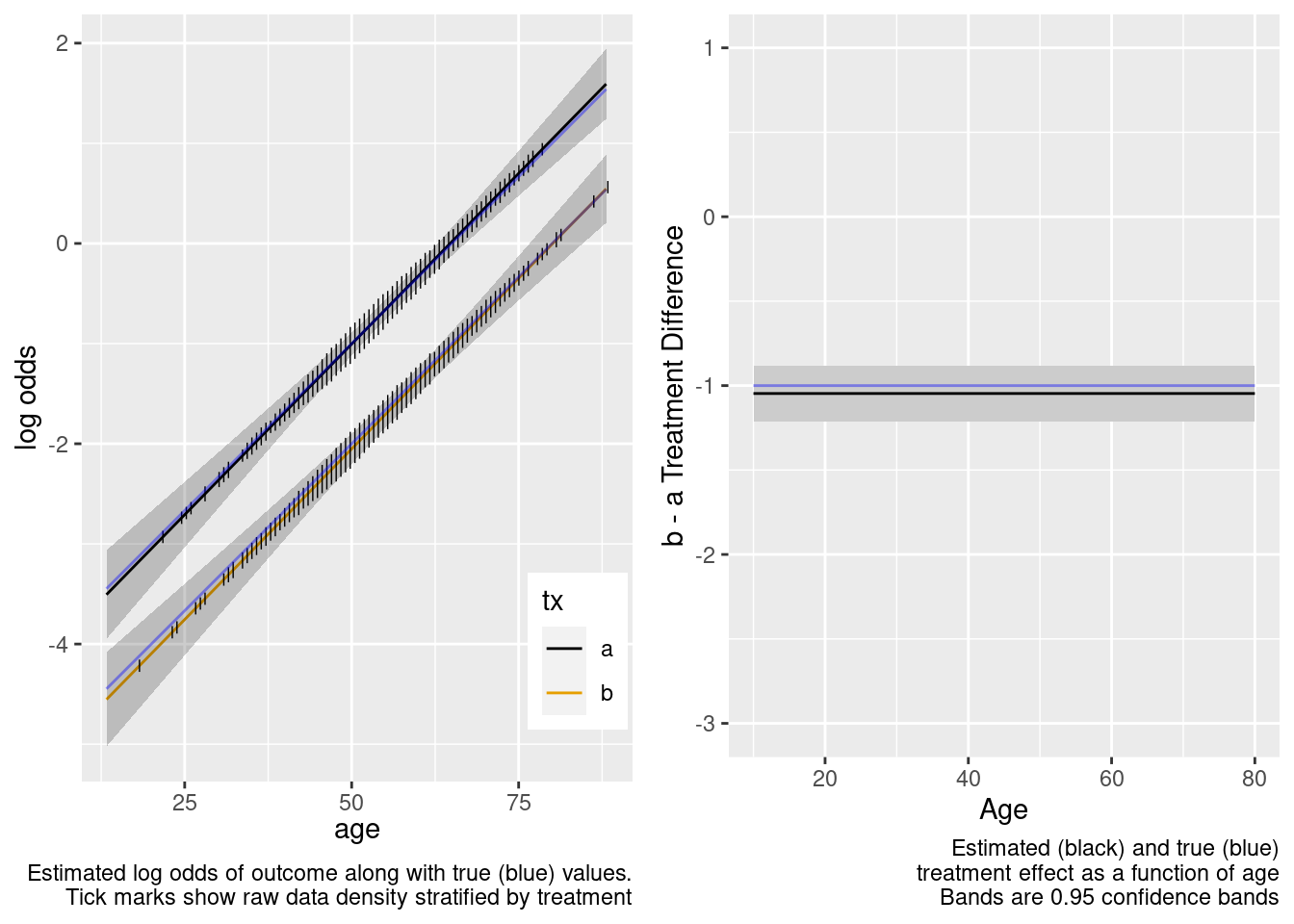This is the correct model.

Logistic Regression Model

``` lrm(formula = y ~ tx * age, data = d)
```

Frequencies of Missing Values Due to Each Variable

```    y   tx  age
1444    0    0
```
Model Likelihood
Ratio Test
Discrimination
Indexes
Rank Discrim.
Indexes
Obs 3556 LR χ2 330.73 R2 0.131 C 0.697
0 2658 d.f. 3 R23,3556 0.088 Dxy 0.394
1 898 Pr(>χ2) <0.0001 R23,2013.7 0.150 γ 0.394
max |∂log L/∂β| 1×10-11 Brier 0.171 τa 0.149
β S.E. Wald Z Pr(>|Z|)
Intercept  -4.6606  0.3849 -12.11 <0.0001
tx=b  -0.4360  0.6056 -0.72 0.4715
age   0.0726  0.0069 10.56 <0.0001
tx=b × age  -0.0108  0.0106 -1.02 0.3089
 χ2 d.f. P Wald Statistics for `y` tx (Factor+Higher Order Factors) 154.98 2 <0.0001 All Interactions 1.04 1 0.3089 age (Factor+Higher Order Factors) 169.90 2 <0.0001 All Interactions 1.04 1 0.3089 tx × age (Factor+Higher Order Factors) 1.04 1 0.3089 TOTAL 292.05 3 <0.0001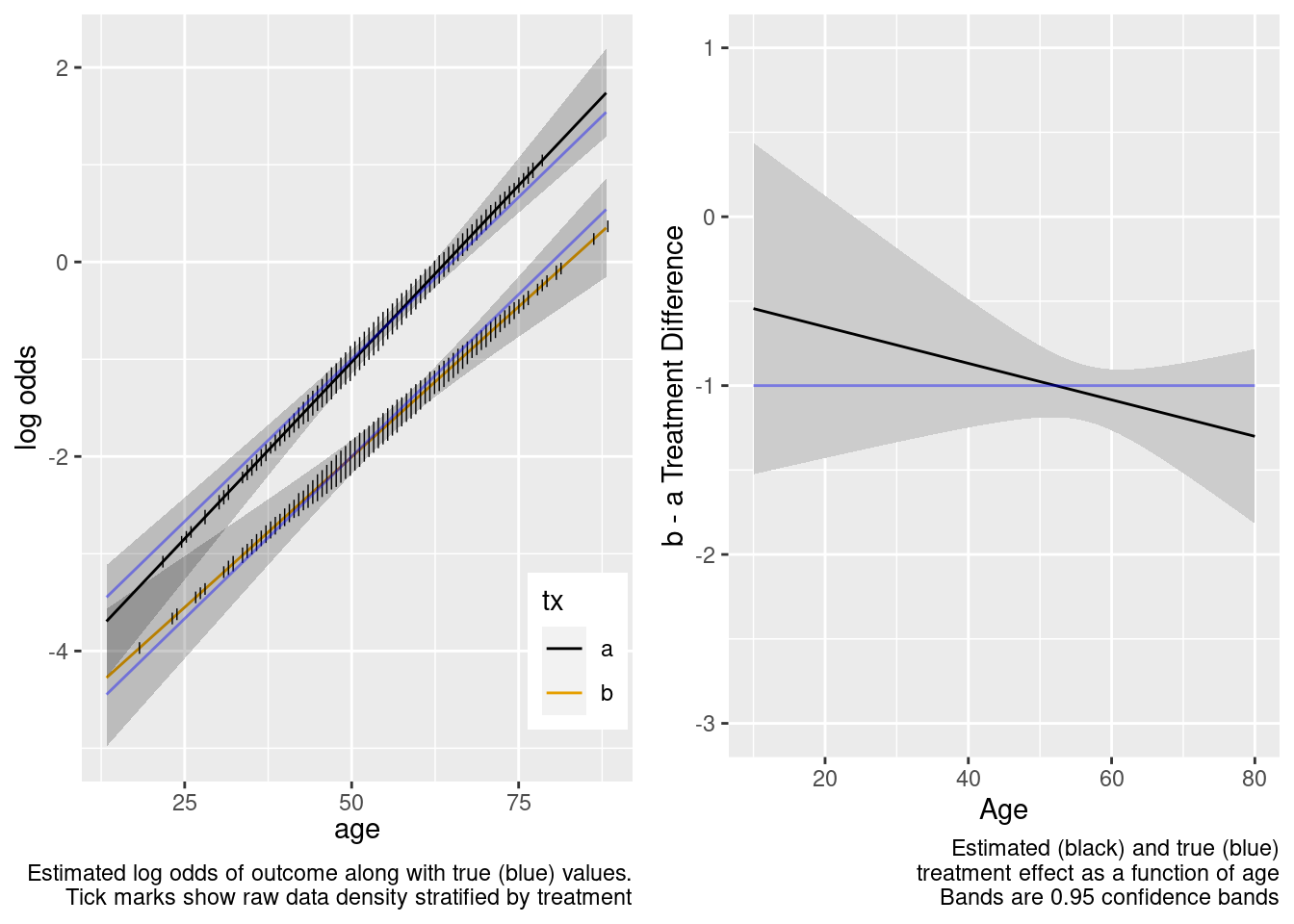This model included an unnecessary linear interaction term.

Logistic Regression Model

``` lrm(formula = form, data = d, tol = 1e-12)
```

Frequencies of Missing Values Due to Each Variable

```    y   tx  age
1444    0    0
```
Model Likelihood
Ratio Test
Discrimination
Indexes
Rank Discrim.
Indexes
Obs 3556 LR χ2 333.43 R2 0.132 C 0.697
0 2658 d.f. 7 R27,3556 0.088 Dxy 0.395
1 898 Pr(>χ2) <0.0001 R27,2013.7 0.150 γ 0.395
max |∂log L/∂β| 5×10-11 Brier 0.171 τa 0.149
β S.E. Wald Z Pr(>|Z|)
Intercept  -4.7933  0.5949 -8.06 <0.0001
tx=b   0.6350  0.9700 0.65 0.5127
age   0.0752  0.0113 6.67 <0.0001
age’  -0.0274  0.1673 -0.16 0.8699
age’’   0.0392  0.4011 0.10 0.9221
tx=b × age  -0.0319  0.0183 -1.74 0.0820
tx=b × age’   0.2854  0.2321 1.23 0.2188
tx=b × age’’  -0.5634  0.5254 -1.07 0.2836
 χ2 d.f. P Wald Statistics for `y` tx (Factor+Higher Order Factors) 155.86 4 <0.0001 All Interactions 3.03 3 0.3873 age (Factor+Higher Order Factors) 175.89 6 <0.0001 All Interactions 3.03 3 0.3873 Nonlinear (Factor+Higher Order Factors) 2.73 4 0.6041 tx × age (Factor+Higher Order Factors) 3.03 3 0.3873 Nonlinear 1.93 2 0.3813 Nonlinear Interaction : f(A,B) vs. AB 1.93 2 0.3813 TOTAL NONLINEAR 2.73 4 0.6041 TOTAL NONLINEAR + INTERACTION 3.82 5 0.5755 TOTAL 299.44 7 <0.0001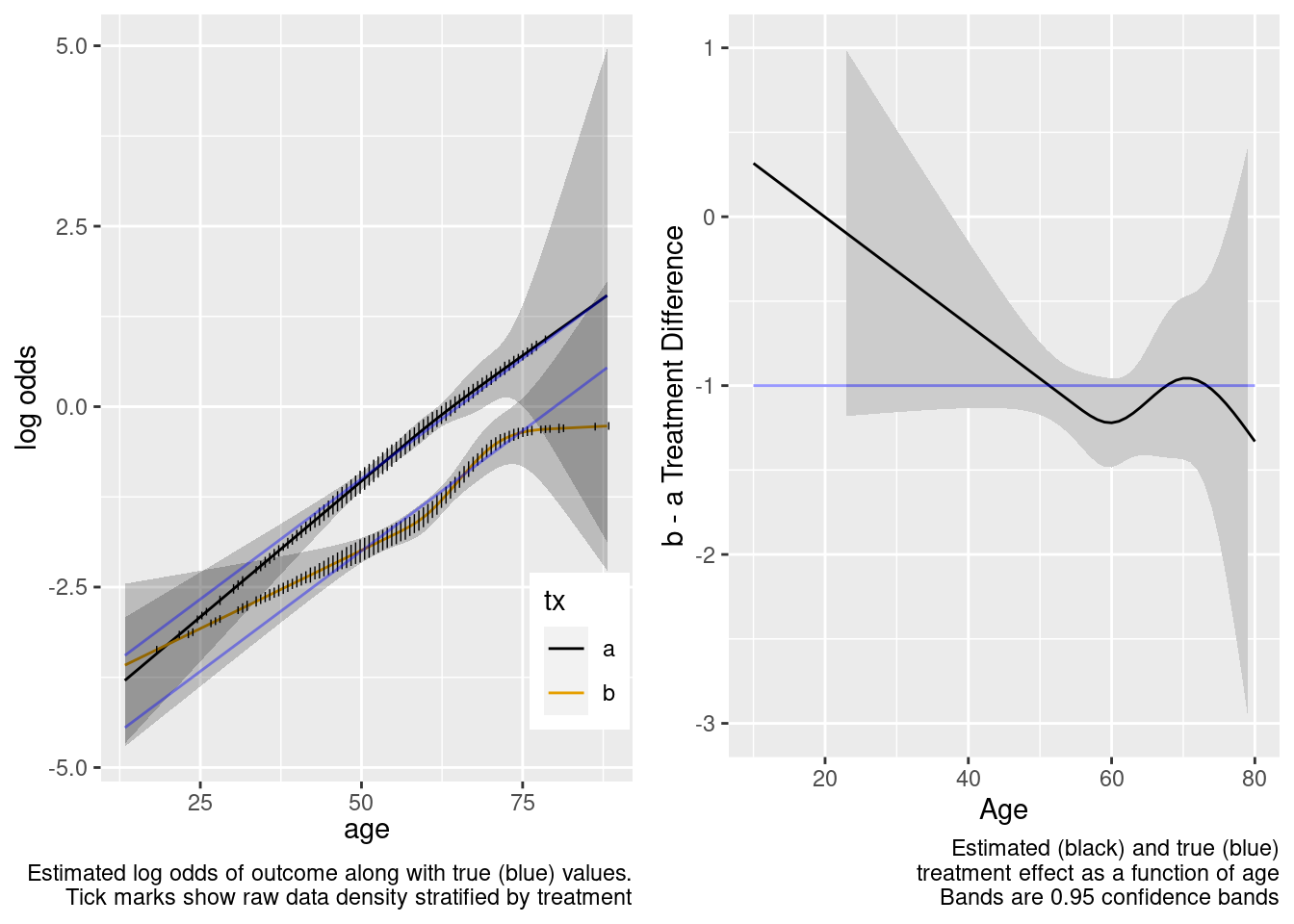This model included unnecessary linear and nonlinear interaction terms.

## Case Where Treatment Truly Interacts with Age

### Estimation of Treatment Effect in Population With No Overlap

Next turn to the case where the true data generating model has a linear treatment by age interaction which we may or may not include in our model. The true model has a treatment effect of -1.0 for age 50 patients, and each year below 50 results in a further reduction by 0.03 in the effect.

Logistic Regression Model

``` lrm(formula = y ~ tx + age, data = d)
```

Frequencies of Missing Values Due to Each Variable

```    y   tx  age
2539    0    0
```
Model Likelihood
Ratio Test
Discrimination
Indexes
Rank Discrim.
Indexes
Obs 2461 LR χ2 257.32 R2 0.144 C 0.701
0 1787 d.f. 2 R22,2461 0.099 Dxy 0.403
1 674 Pr(>χ2) <0.0001 R22,1468.2 0.160 γ 0.403
max |∂log L/∂β| 9×10-6 Brier 0.178 τa 0.160
β S.E. Wald Z Pr(>|Z|)
Intercept  -3.8181  0.4509 -8.47 <0.0001
tx=b  -1.4027  0.1020 -13.76 <0.0001
age   0.0583  0.0077 7.61 <0.0001
 χ2 d.f. P Wald Statistics for `y` tx 189.24 1 <0.0001 age 57.92 1 <0.0001 TOTAL 223.45 2 <0.0001This model failed to include a needed interaction term.

Logistic Regression Model

``` lrm(formula = y ~ tx * age, data = d)
```

Frequencies of Missing Values Due to Each Variable

```    y   tx  age
2539    0    0
```
Model Likelihood
Ratio Test
Discrimination
Indexes
Rank Discrim.
Indexes
Obs 2461 LR χ2 258.51 R2 0.144 C 0.702
0 1787 d.f. 3 R23,2461 0.099 Dxy 0.403
1 674 Pr(>χ2) <0.0001 R23,1468.2 0.160 γ 0.403
max |∂log L/∂β| 2×10-12 Brier 0.178 τa 0.160
β S.E. Wald Z Pr(>|Z|)
Intercept  -4.2193  0.5852 -7.21 <0.0001
tx=b  -0.3879  0.9367 -0.41 0.6788
age   0.0652  0.0100 6.53 <0.0001
tx=b × age  -0.0171  0.0157 -1.09 0.2765
 χ2 d.f. P Wald Statistics for `y` tx (Factor+Higher Order Factors) 191.47 2 <0.0001 All Interactions 1.18 1 0.2765 age (Factor+Higher Order Factors) 58.35 2 <0.0001 All Interactions 1.18 1 0.2765 tx × age (Factor+Higher Order Factors) 1.18 1 0.2765 TOTAL 228.30 3 <0.0001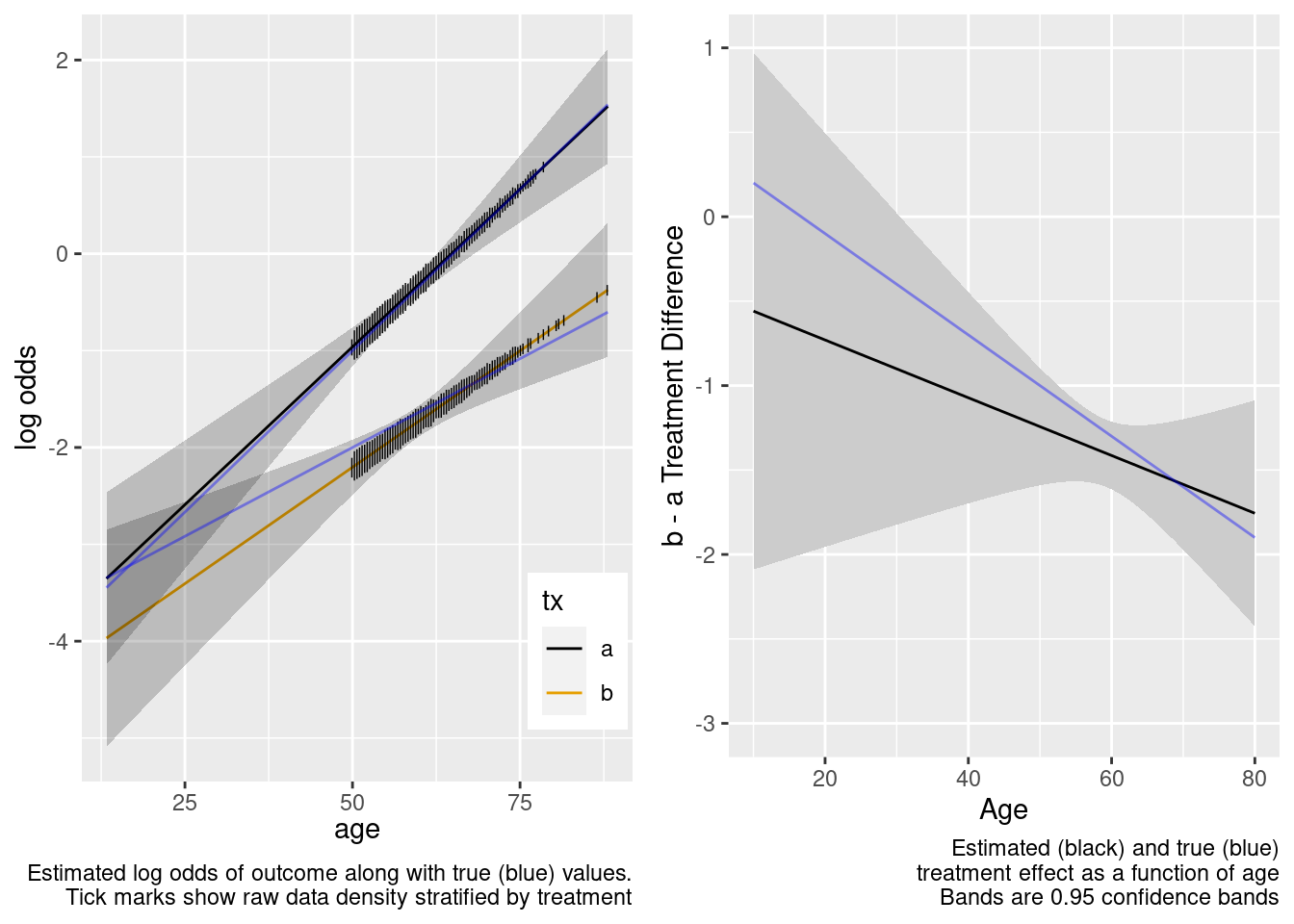Because of the more limited age range in the trial there was insufficient power to provide definitive statistical evidence for an interaction, but the point estimate for the interaction effect is not unreasonable. Though confidence bands are wide because of no overlap, the extrapolated treatment effects are reasonable as a result.

This is the correct model.

Logistic Regression Model

``` lrm(formula = form, data = d, tol = 1e-12)
```

Frequencies of Missing Values Due to Each Variable

```    y   tx  age
2539    0    0
```
Model Likelihood
Ratio Test
Discrimination
Indexes
Rank Discrim.
Indexes
Obs 2461 LR χ2 261.24 R2 0.146 C 0.702
0 1787 d.f. 7 R27,2461 0.098 Dxy 0.403
1 674 Pr(>χ2) <0.0001 R27,1468.2 0.159 γ 0.404
max |∂log L/∂β| 1×10-12 Brier 0.178 τa 0.161
β S.E. Wald Z Pr(>|Z|)
Intercept  -3.0988  1.2779 -2.42 0.0153
tx=b   0.1305  2.1946 0.06 0.9526
age   0.0445  0.0231 1.93 0.0542
age’   0.2124  0.2213 0.96 0.3372
age’’  -0.4384  0.4906 -0.89 0.3716
tx=b × age  -0.0267  0.0396 -0.68 0.4996
tx=b × age’   0.0955  0.3313 0.29 0.7731
tx=b × age’’  -0.1934  0.6939 -0.28 0.7805
 χ2 d.f. P Wald Statistics for `y` tx (Factor+Higher Order Factors) 191.14 4 <0.0001 All Interactions 1.19 3 0.7545 age (Factor+Higher Order Factors) 61.60 6 <0.0001 All Interactions 1.19 3 0.7545 Nonlinear (Factor+Higher Order Factors) 2.67 4 0.6150 tx × age (Factor+Higher Order Factors) 1.19 3 0.7545 Nonlinear 0.08 2 0.9590 Nonlinear Interaction : f(A,B) vs. AB 0.08 2 0.9590 TOTAL NONLINEAR 2.67 4 0.6150 TOTAL NONLINEAR + INTERACTION 3.78 5 0.5816 TOTAL 229.68 7 <0.0001This model correctly captures linear interaction, but also allows for unnecessary nonlinear interaction.

### RCT Sample Partially Overlaps with Target Population

Sticking with the true treatment effect interacting with age, we generate data with the same partial overlap as before, and repeat the same analyses. We start by fitting a model that is oblivious to interaction.

Logistic Regression Model

``` lrm(formula = y ~ tx + age, data = d)
```

Frequencies of Missing Values Due to Each Variable

```    y   tx  age
1444    0    0
```
Model Likelihood
Ratio Test
Discrimination
Indexes
Rank Discrim.
Indexes
Obs 3556 LR χ2 323.95 R2 0.130 C 0.699
0 2702 d.f. 2 R22,3556 0.087 Dxy 0.397
1 854 Pr(>χ2) <0.0001 R22,1946.7 0.152 γ 0.397
max |∂log L/∂β| 8×10-12 Brier 0.165 τa 0.145
β S.E. Wald Z Pr(>|Z|)
Intercept  -3.7735  0.2939 -12.84 <0.0001
tx=b  -1.2305  0.0876 -14.04 <0.0001
age   0.0566  0.0052 10.80 <0.0001
 χ2 d.f. P Wald Statistics for `y` tx 197.20 1 <0.0001 age 116.73 1 <0.0001 TOTAL 281.79 2 <0.0001This model failed to include a needed interaction term.

Logistic Regression Model

``` lrm(formula = y ~ tx * age, data = d)
```

Frequencies of Missing Values Due to Each Variable

```    y   tx  age
1444    0    0
```
Model Likelihood
Ratio Test
Discrimination
Indexes
Rank Discrim.
Indexes
Obs 3556 LR χ2 338.39 R2 0.136 C 0.700
0 2702 d.f. 3 R23,3556 0.090 Dxy 0.400
1 854 Pr(>χ2) <0.0001 R23,1946.7 0.158 γ 0.401
max |∂log L/∂β| 3×10-12 Brier 0.164 τa 0.146
β S.E. Wald Z Pr(>|Z|)
Intercept  -4.6606  0.3849 -12.11 <0.0001
tx=b   1.0820  0.6129 1.77 0.0775
age   0.0726  0.0069 10.56 <0.0001
tx=b × age  -0.0412  0.0109 -3.79 0.0002
 χ2 d.f. P Wald Statistics for `y` tx (Factor+Higher Order Factors) 210.59 2 <0.0001 All Interactions 14.36 1 0.0002 age (Factor+Higher Order Factors) 125.47 2 <0.0001 All Interactions 14.36 1 0.0002 tx × age (Factor+Higher Order Factors) 14.36 1 0.0002 TOTAL 310.44 3 <0.0001The amount of interaction estimated from the larger older sample extrapolated well to the younger target population.

This is the correct model.

Logistic Regression Model

``` lrm(formula = form, data = d, tol = 1e-12)
```

Frequencies of Missing Values Due to Each Variable

```    y   tx  age
1444    0    0
```
Model Likelihood
Ratio Test
Discrimination
Indexes
Rank Discrim.
Indexes
Obs 3556 LR χ2 343.94 R2 0.138 C 0.700
0 2702 d.f. 7 R27,3556 0.090 Dxy 0.401
1 854 Pr(>χ2) <0.0001 R27,1946.7 0.159 γ 0.401
max |∂log L/∂β| 7×10-12 Brier 0.164 τa 0.146
β S.E. Wald Z Pr(>|Z|)
Intercept  -4.7933  0.5949 -8.06 <0.0001
tx=b   2.5181  0.9368 2.69 0.0072
age   0.0752  0.0113 6.67 <0.0001
age’  -0.0274  0.1673 -0.16 0.8699
age’’   0.0392  0.4011 0.10 0.9221
tx=b × age  -0.0698  0.0179 -3.91 <0.0001
tx=b × age’   0.4148  0.2398 1.73 0.0836
tx=b × age’’  -0.8163  0.5469 -1.49 0.1355
 χ2 d.f. P Wald Statistics for `y` tx (Factor+Higher Order Factors) 211.50 4 <0.0001 All Interactions 19.06 3 0.0003 age (Factor+Higher Order Factors) 132.65 6 <0.0001 All Interactions 19.06 3 0.0003 Nonlinear (Factor+Higher Order Factors) 5.66 4 0.2263 tx × age (Factor+Higher Order Factors) 19.06 3 0.0003 Nonlinear 3.97 2 0.1374 Nonlinear Interaction : f(A,B) vs. AB 3.97 2 0.1374 TOTAL NONLINEAR 5.66 4 0.2263 TOTAL NONLINEAR + INTERACTION 20.91 5 0.0008 TOTAL 316.28 7 <0.0001The spline interaction estimates did not extrapolate well to the very young.

This model correctly captures linear interaction, but also allows for unnecessary nonlinear interaction.

# Ability to Compare Treatments in Observational Studies

When a categorical baseline characteristic has all of the patients in one of its categories getting a single treatment, one cannot estimate efficacy in that category unless (1) there is no interaction between treatment and the variable or (2) the baseline variable does not relate to outcome. If one of these conditions is not satisfied, one would have to do a conditional analysis, i.e., to estimate efficacy in patients not in the offending category. If a continuous baseline variable has an interval for which every patient in that interval received only one treatment, then one cannot estimate the treatment effect in that interval unless (1) there is no interaction (linear or nonlinear) between the variable and treatment or (2) the variable is irrelevant to outcome. So when you hear that non-overlap implies that only a conditional treatment comparison can be done, be aware of the true underlying assumptions. Note that non-overlap on a variable implies non-overlap for one or more values of a propensity score.

To illustrate these points, consider an observational study where the data generating process is from the same population logistic model as used above, but instead of considering generalizability to a population, consider the in-sample comparison of treatments a and b adjusted for age. We start with a sample in which all those with age ≥ 50 got treatment b and all those with age < 50 got treatment a.

## True Model Has No Treatment Interactions

### No Overlap Between Treatment Groups

All patients age < 50 had treatment a, and those ≥ 50 had b.

Age distributions in the observed treatment groups are shown below.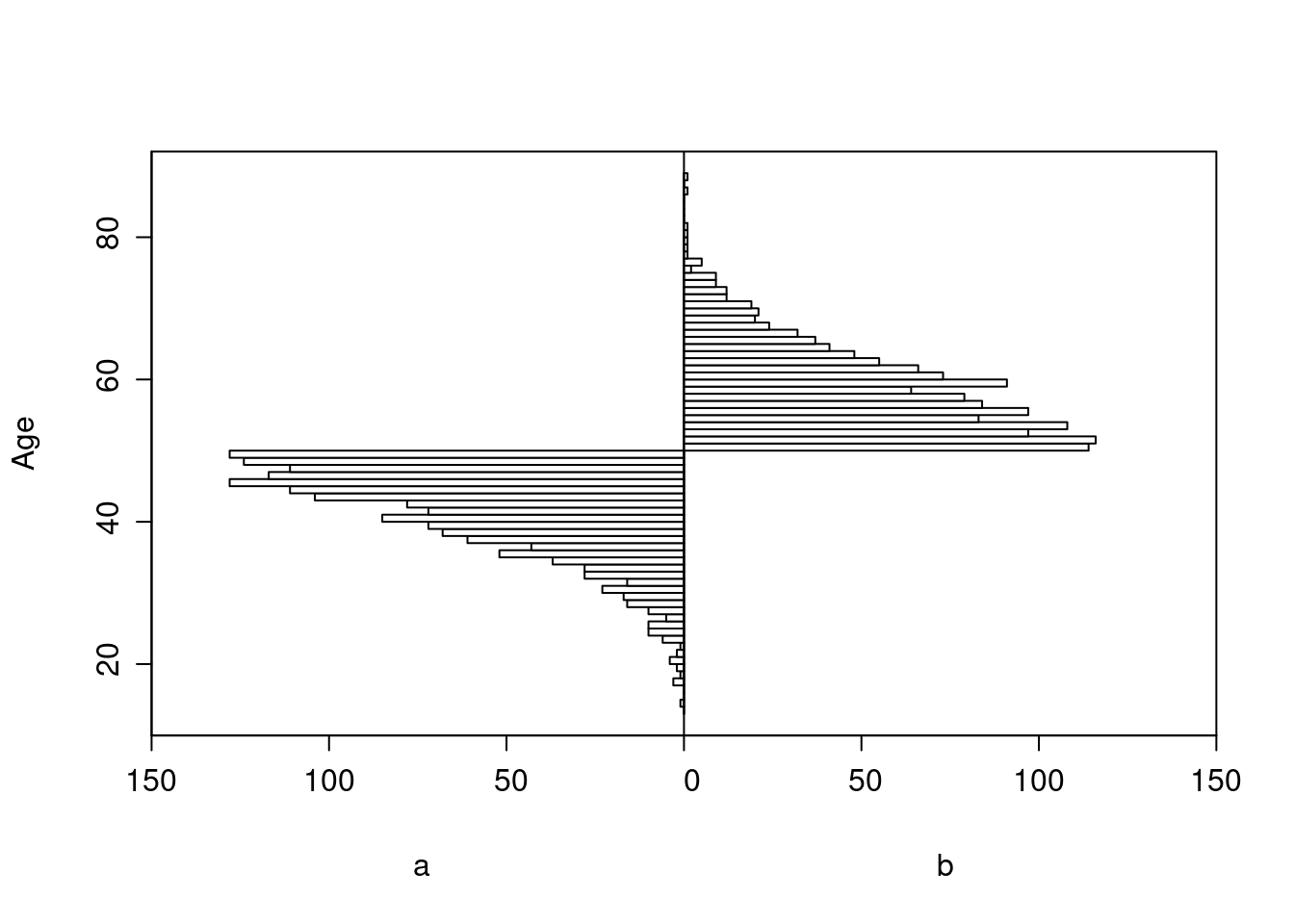Logistic Regression Model

``` lrm(formula = y ~ tx + age, data = d)
```
Model Likelihood
Ratio Test
Discrimination
Indexes
Rank Discrim.
Indexes
Obs 2999 LR χ2 87.42 R2 0.046 C 0.616
0 2431 d.f. 2 R22,2999 0.028 Dxy 0.232
1 568 Pr(>χ2) <0.0001 R22,1381.3 0.060 γ 0.232
max |∂log L/∂β| 8×10-9 Brier 0.149 τa 0.071
β S.E. Wald Z Pr(>|Z|)
Intercept  -4.5743  0.3532 -12.95 <0.0001
tx=b  -1.0283  0.1608 -6.40 <0.0001
age   0.0714  0.0080 8.96 <0.0001
 χ2 d.f. P Wald Statistics for `y` tx 40.90 1 <0.0001 age 80.26 1 <0.0001 TOTAL 82.33 2 <0.0001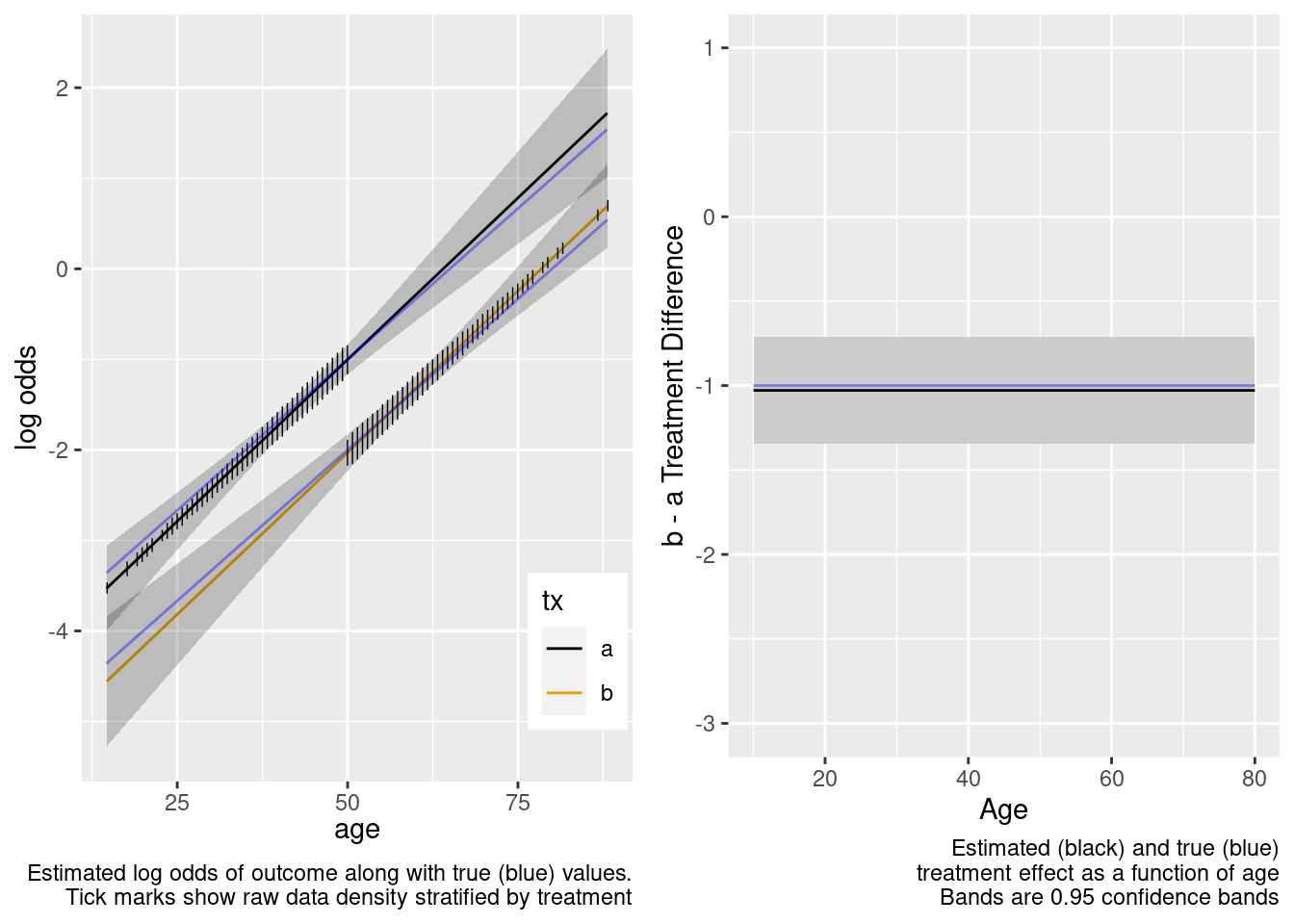The model-based covariate adjustment provided the correct estimate of the treatment effect even with no overlap, since the specified model is the correct model.

This is the correct model.

Logistic Regression Model

``` lrm(formula = y ~ tx * age, data = d)
```
Model Likelihood
Ratio Test
Discrimination
Indexes
Rank Discrim.
Indexes
Obs 2999 LR χ2 88.00 R2 0.047 C 0.616
0 2431 d.f. 3 R23,2999 0.028 Dxy 0.231
1 568 Pr(>χ2) <0.0001 R23,1381.3 0.060 γ 0.232
max |∂log L/∂β| 5×10-7 Brier 0.149 τa 0.071
β S.E. Wald Z Pr(>|Z|)
Intercept  -4.2550  0.5399 -7.88 <0.0001
tx=b  -1.6471  0.8237 -2.00 0.0455
age   0.0641  0.0124 5.19 <0.0001
tx=b × age   0.0124  0.0161 0.77 0.4430
 χ2 d.f. P Wald Statistics for `y` tx (Factor+Higher Order Factors) 40.98 2 <0.0001 All Interactions 0.59 1 0.4430 age (Factor+Higher Order Factors) 81.73 2 <0.0001 All Interactions 0.59 1 0.4430 tx × age (Factor+Higher Order Factors) 0.59 1 0.4430 TOTAL 84.33 3 <0.0001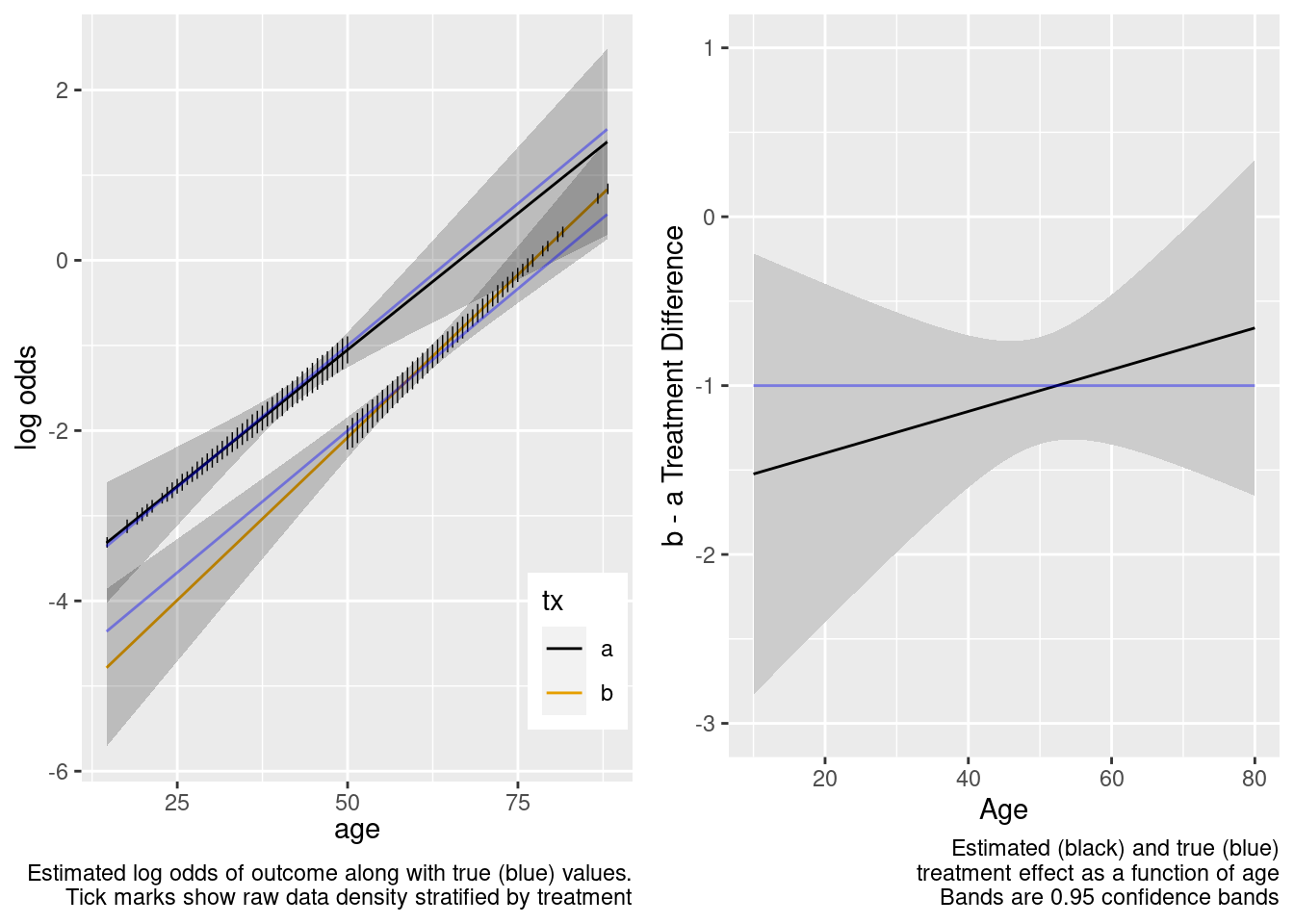With no age overlap, the treatment by age interaction is estimated very inefficiently and in this case incorrectly. Wide confidence bands correctly capture the difficulty of the task of estimating age-specific treatment effects.

This model included an unnecessary linear interaction term.

Logistic Regression Model

``` lrm(formula = form, data = d, tol = 1e-12)
```
Model Likelihood
Ratio Test
Discrimination
Indexes
Rank Discrim.
Indexes
Obs 2999 LR χ2 89.34 R2 0.047 C 0.617
0 2431 d.f. 5 R25,2999 0.028 Dxy 0.233
1 568 Pr(>χ2) <0.0001 R25,1381.3 0.059 γ 0.234
max |∂log L/∂β| 7×10-12 Brier 0.149 τa 0.072
β S.E. Wald Z Pr(>|Z|)
Intercept  -5.0833  0.9349 -5.44 <0.0001
tx=b   0.4348  7.1719 0.06 0.9517
age   0.0868  0.0241 3.60 0.0003
age’  -0.1233  0.1090 -1.13 0.2580
tx=b × age  -0.0361  0.1482 -0.24 0.8073
tx=b × age’   0.1420  0.1520 0.93 0.3502
 χ2 d.f. P Wald Statistics for `y` tx (Factor+Higher Order Factors) 40.98 3 <0.0001 All Interactions 1.50 2 0.4734 age (Factor+Higher Order Factors) 80.92 4 <0.0001 All Interactions 1.50 2 0.4734 Nonlinear (Factor+Higher Order Factors) 1.31 2 0.5193 tx × age (Factor+Higher Order Factors) 1.50 2 0.4734 Nonlinear 0.87 1 0.3502 Nonlinear Interaction : f(A,B) vs. AB 0.87 1 0.3502 TOTAL NONLINEAR 1.31 2 0.5193 TOTAL NONLINEAR + INTERACTION 1.90 3 0.5925 TOTAL 83.33 5 <0.0001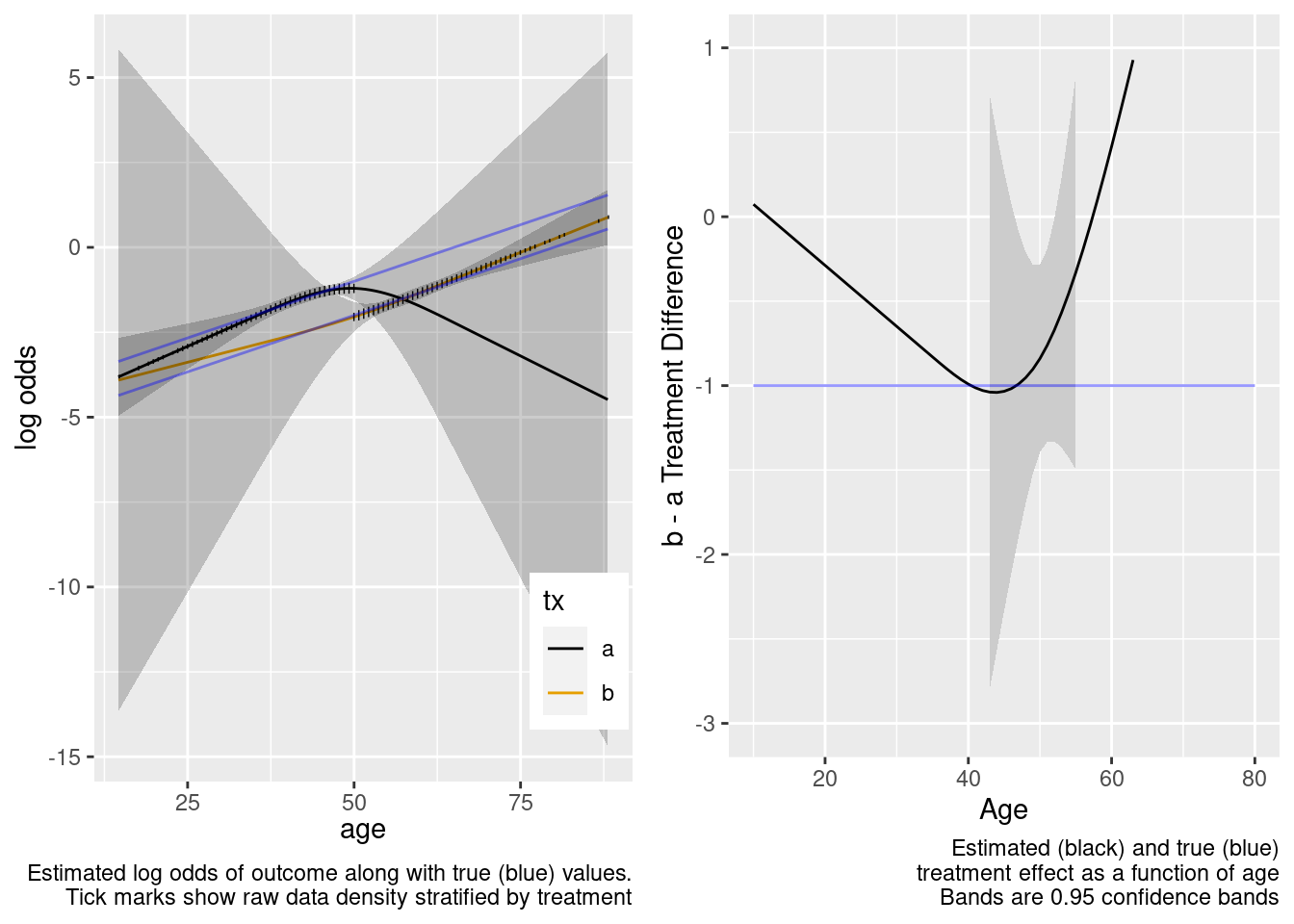This model included unnecessary linear and nonlinear interaction terms.

### Some Overlap in Ages Between Treatments

Now consider an observational study, again with confounding by indication, related to age. Partial overlap is defined by specifying separate study inclusion probability functions. Given the age and treatment these functions specify the probability that a patient would be included in the observational study. The first plot shows the inclusion probabilities separately for treatments a and b. Then the result of a linear no-interaction model are shown. As before, blue curves depict true data-generating model log odds.

The graph below shows the probability of selection into each of the observed treatment groups as a function of age.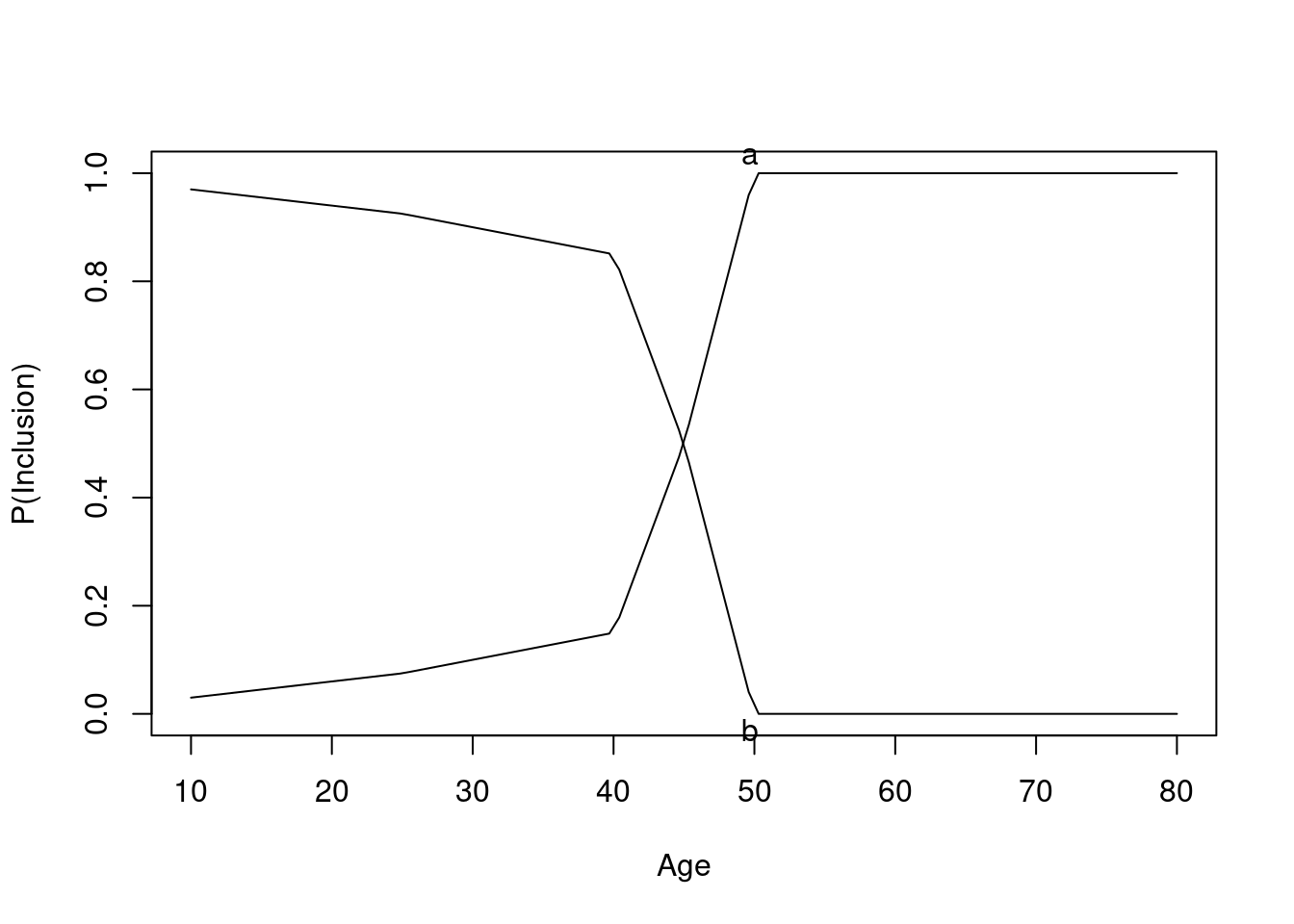Age distributions in the observed treatment groups are shown below.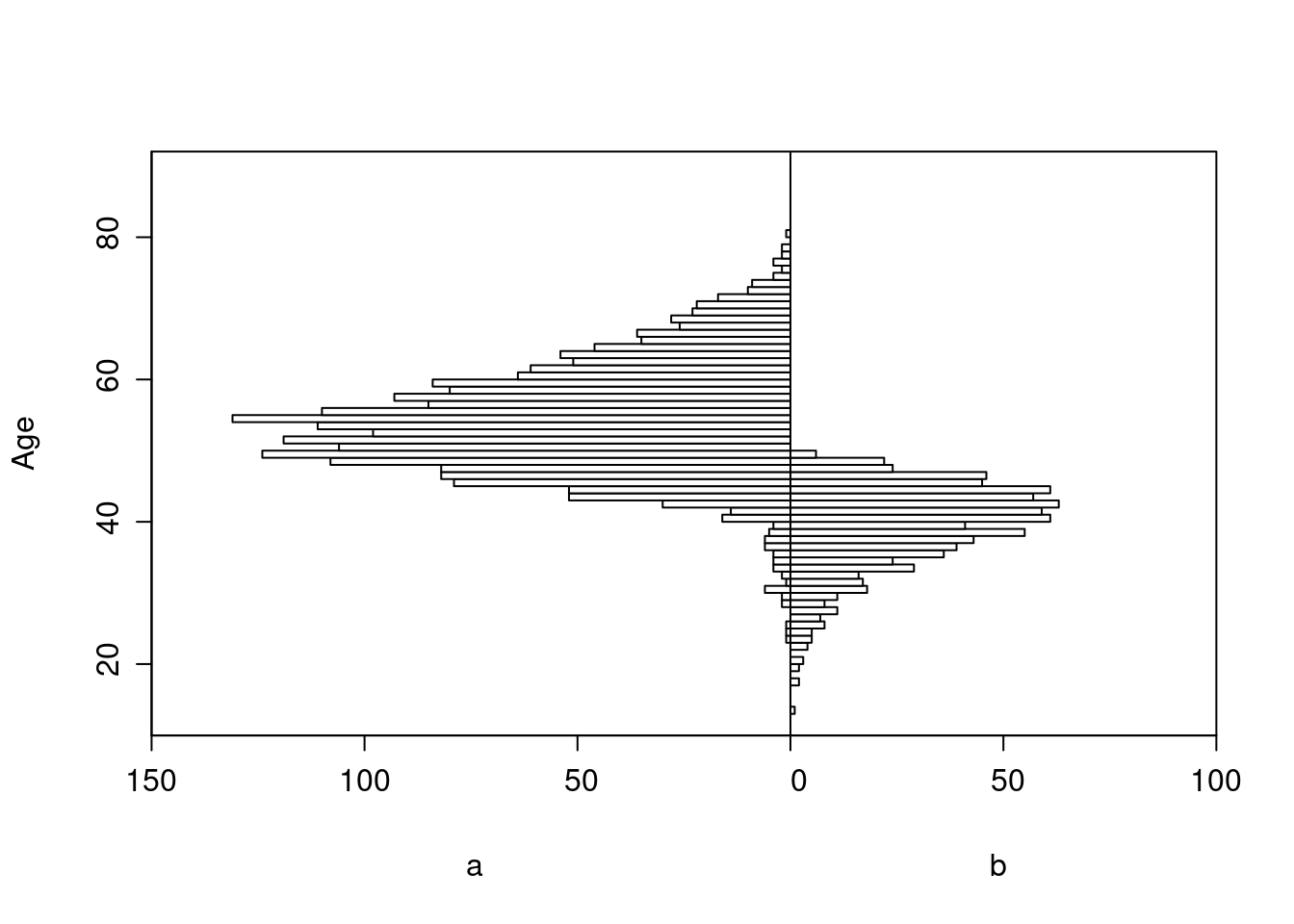Logistic Regression Model

``` lrm(formula = y ~ tx + age, data = d)
```
Model Likelihood
Ratio Test
Discrimination
Indexes
Rank Discrim.
Indexes
Obs 3031 LR χ2 474.99 R2 0.212 C 0.744
0 2236 d.f. 2 R22,3031 0.144 Dxy 0.488
1 795 Pr(>χ2) <0.0001 R22,1759.4 0.236 γ 0.489
max |∂log L/∂β| 9×10-6 Brier 0.166 τa 0.189
β S.E. Wald Z Pr(>|Z|)
Intercept  -4.8959  0.3443 -14.22 <0.0001
tx=b  -1.0104  0.1787 -5.65 <0.0001
age   0.0769  0.0061 12.53 <0.0001
 χ2 d.f. P Wald Statistics for `y` tx 31.98 1 <0.0001 age 156.93 1 <0.0001 TOTAL 335.25 2 <0.0001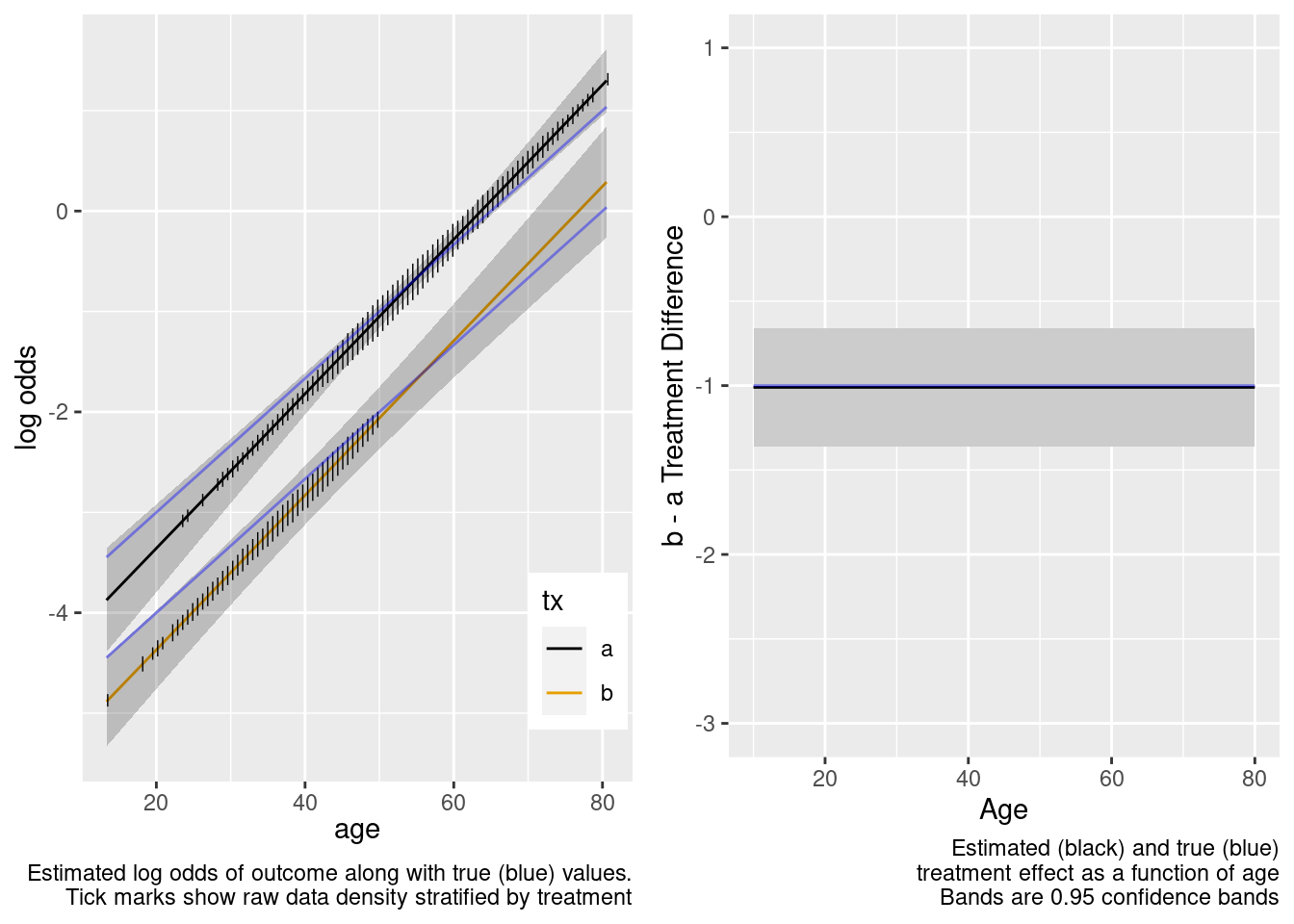This is the correct model.

Logistic Regression Model

``` lrm(formula = y ~ tx * age, data = d)
```
Model Likelihood
Ratio Test
Discrimination
Indexes
Rank Discrim.
Indexes
Obs 3031 LR χ2 475.20 R2 0.212 C 0.744
0 2236 d.f. 3 R23,3031 0.144 Dxy 0.488
1 795 Pr(>χ2) <0.0001 R23,1759.4 0.235 γ 0.489
max |∂log L/∂β| 1×10-8 Brier 0.166 τa 0.189
β S.E. Wald Z Pr(>|Z|)
Intercept  -4.8638  0.3512 -13.85 <0.0001
tx=b  -1.6044  1.3432 -1.19 0.2323
age   0.0763  0.0063 12.18 <0.0001
tx=b × age   0.0141  0.0314 0.45 0.6546
 χ2 d.f. P Wald Statistics for `y` tx (Factor+Higher Order Factors) 32.33 2 <0.0001 All Interactions 0.20 1 0.6546 age (Factor+Higher Order Factors) 157.04 2 <0.0001 All Interactions 0.20 1 0.6546 tx × age (Factor+Higher Order Factors) 0.20 1 0.6546 TOTAL 330.68 3 <0.0001The confidence bands are correctly registering that there is little information about the treatment effect outside of the heavier overlap region.

This model included an unnecessary linear interaction term.

Logistic Regression Model

``` lrm(formula = form, data = d, tol = 1e-12)
```
Model Likelihood
Ratio Test
Discrimination
Indexes
Rank Discrim.
Indexes
Obs 3031 LR χ2 476.54 R2 0.213 C 0.744
0 2236 d.f. 5 R25,3031 0.144 Dxy 0.489
1 795 Pr(>χ2) <0.0001 R25,1759.4 0.235 γ 0.489
max |∂log L/∂β| 2×10-7 Brier 0.166 τa 0.189
β S.E. Wald Z Pr(>|Z|)
Intercept  -6.2165  1.3037 -4.77 <0.0001
tx=b   0.2424  2.3347 0.10 0.9173
age   0.1058  0.0280 3.78 0.0002
age’  -0.0262  0.0240 -1.09 0.2761
tx=b × age  -0.0291  0.0579 -0.50 0.6155
tx=b × age’   0.1247  0.2974 0.42 0.6749
 χ2 d.f. P Wald Statistics for `y` tx (Factor+Higher Order Factors) 20.25 3 0.0002 All Interactions 0.26 2 0.8794 age (Factor+Higher Order Factors) 154.87 4 <0.0001 All Interactions 0.26 2 0.8794 Nonlinear (Factor+Higher Order Factors) 1.30 2 0.5229 tx × age (Factor+Higher Order Factors) 0.26 2 0.8794 Nonlinear 0.18 1 0.6749 Nonlinear Interaction : f(A,B) vs. AB 0.18 1 0.6749 TOTAL NONLINEAR 1.30 2 0.5229 TOTAL NONLINEAR + INTERACTION 1.51 3 0.6793 TOTAL 330.06 5 <0.0001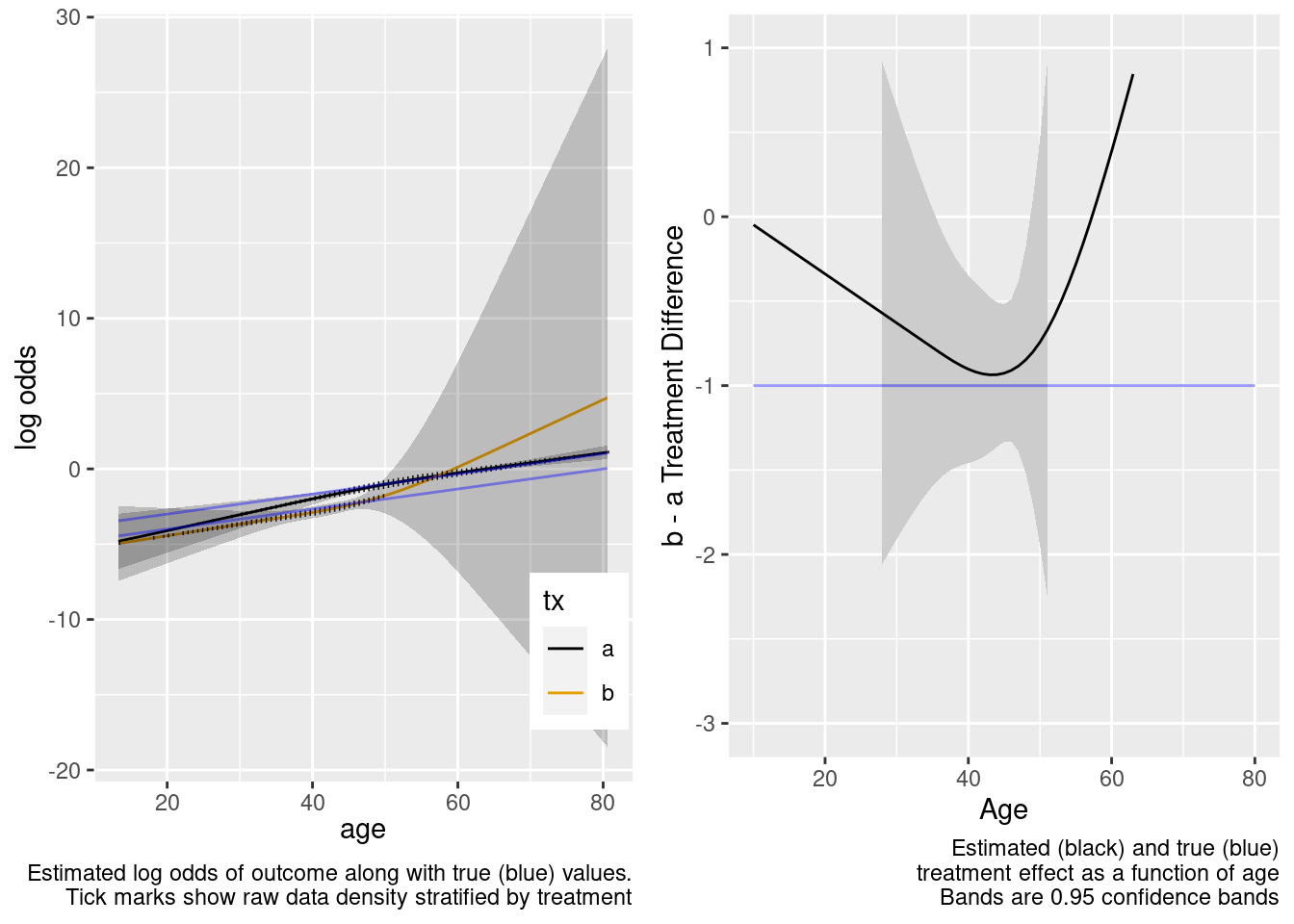This model included unnecessary linear and nonlinear interaction terms.

## Case Where Treatment Truly Interacts with Age

### No Overlap Between Treatment Groups

Return to the data generating model used in the RCT simulation, for which there is truly a linear interaction with treatment, and start with the no overlap case.

Logistic Regression Model

``` lrm(formula = y ~ tx + age, data = d)
```
Model Likelihood
Ratio Test
Discrimination
Indexes
Rank Discrim.
Indexes
Obs 2999 LR χ2 39.09 R2 0.022 C 0.591
0 2493 d.f. 2 R22,2999 0.012 Dxy 0.181
1 506 Pr(>χ2) <0.0001 R22,1261.9 0.029 γ 0.181
max |∂log L/∂β| 4×10-11 Brier 0.139 τa 0.051
β S.E. Wald Z Pr(>|Z|)
Intercept  -3.5659  0.3538 -10.08 <0.0001
tx=b  -0.9499  0.1661 -5.72 <0.0001
age   0.0481  0.0081 5.96 <0.0001
 χ2 d.f. P Wald Statistics for `y` tx 32.71 1 <0.0001 age 35.51 1 <0.0001 TOTAL 37.94 2 <0.0001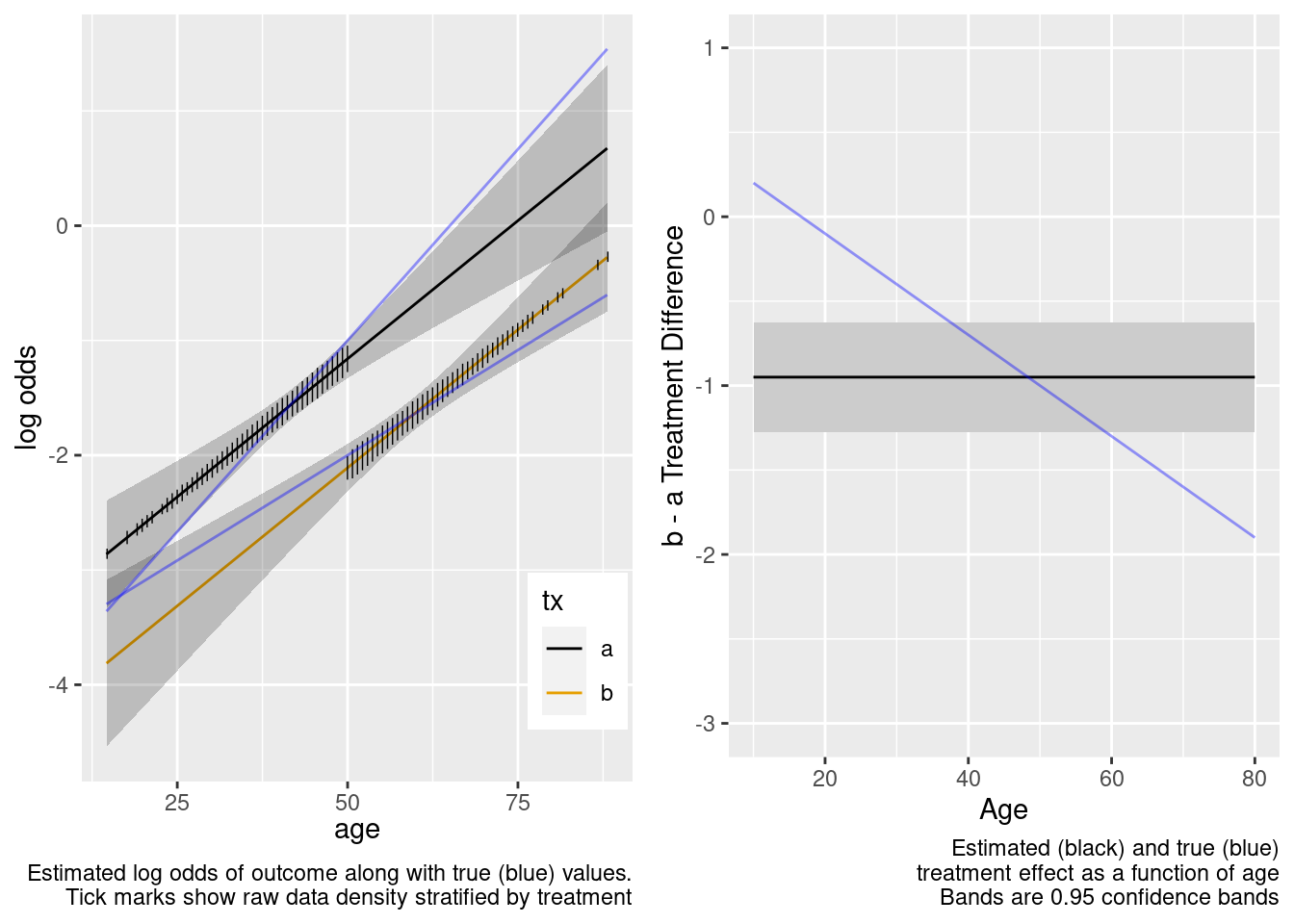The treatment effect is incorrect example at age=50.

This model failed to include a needed interaction term.

Logistic Regression Model

``` lrm(formula = y ~ tx * age, data = d)
```
Model Likelihood
Ratio Test
Discrimination
Indexes
Rank Discrim.
Indexes
Obs 2999 LR χ2 42.28 R2 0.023 C 0.592
0 2493 d.f. 3 R23,2999 0.013 Dxy 0.184
1 506 Pr(>χ2) <0.0001 R23,1261.9 0.031 γ 0.185
max |∂log L/∂β| 3×10-8 Brier 0.138 τa 0.052
β S.E. Wald Z Pr(>|Z|)
Intercept  -4.2550  0.5399 -7.88 <0.0001
tx=b   0.5441  0.8578 0.63 0.5259
age   0.0641  0.0124 5.19 <0.0001
tx=b × age  -0.0295  0.0167 -1.77 0.0767
 χ2 d.f. P Wald Statistics for `y` tx (Factor+Higher Order Factors) 36.26 2 <0.0001 All Interactions 3.13 1 0.0767 age (Factor+Higher Order Factors) 36.45 2 <0.0001 All Interactions 3.13 1 0.0767 tx × age (Factor+Higher Order Factors) 3.13 1 0.0767 TOTAL 40.05 3 <0.0001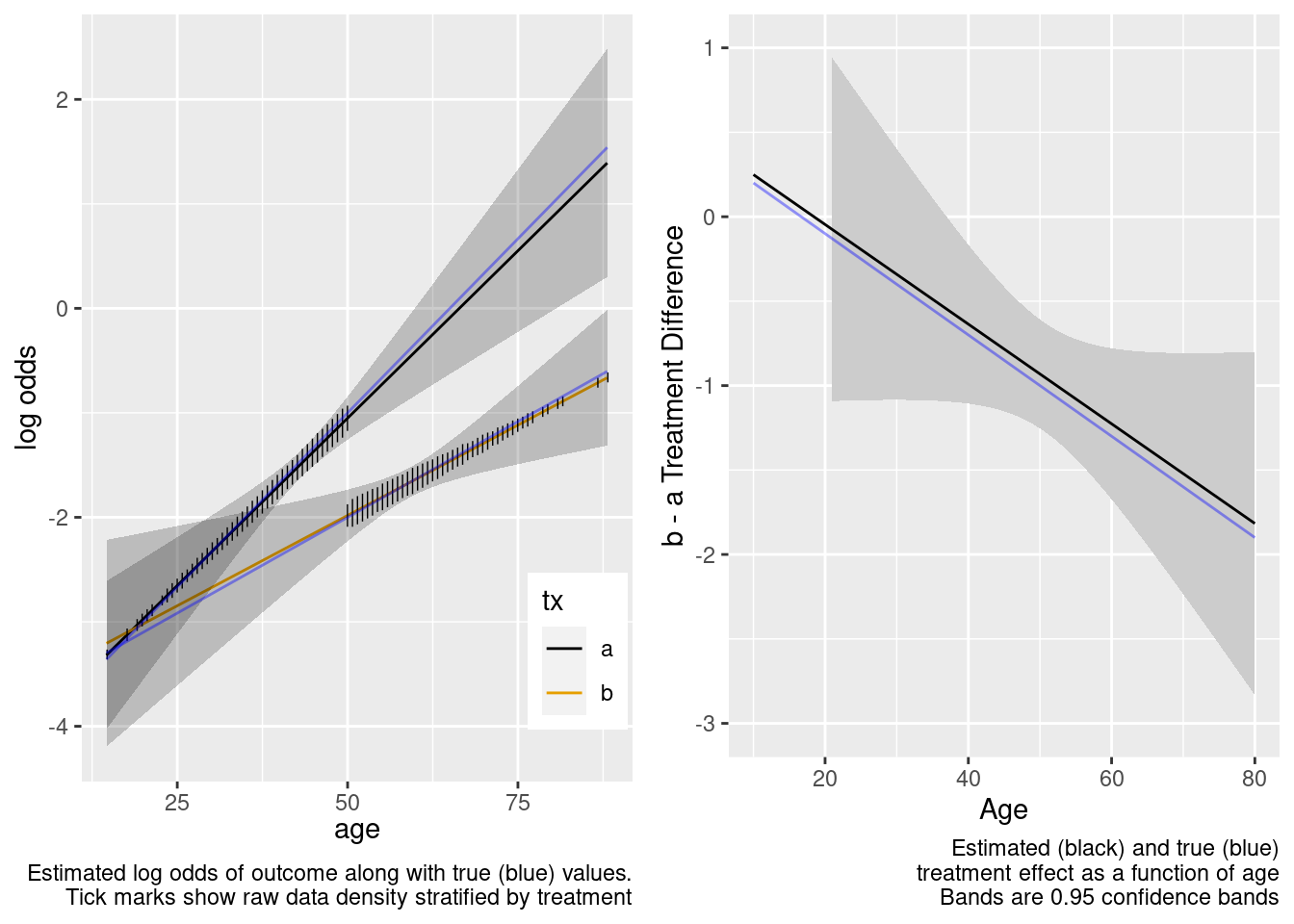The extrapolation is excellent. Now try the overkill model.

This is the correct model.

Logistic Regression Model

``` lrm(formula = form, data = d, tol = 1e-12)
```
Model Likelihood
Ratio Test
Discrimination
Indexes
Rank Discrim.
Indexes
Obs 2999 LR χ2 44.02 R2 0.024 C 0.592
0 2493 d.f. 5 R25,2999 0.013 Dxy 0.184
1 506 Pr(>χ2) <0.0001 R25,1261.9 0.030 γ 0.185
max |∂log L/∂β| 2×10-5 Brier 0.138 τa 0.052
β S.E. Wald Z Pr(>|Z|)
Intercept  -5.0833  0.9349 -5.44 <0.0001
tx=b  -3.5002  7.5833 -0.46 0.6444
age   0.0868  0.0241 3.60 0.0003
age’  -0.1233  0.1090 -1.13 0.2580
tx=b × age   0.0483  0.1568 0.31 0.7581
tx=b × age’   0.0498  0.1569 0.32 0.7508
 χ2 d.f. P Wald Statistics for `y` tx (Factor+Higher Order Factors) 32.65 3 <0.0001 All Interactions 0.97 2 0.6143 age (Factor+Higher Order Factors) 35.52 4 <0.0001 All Interactions 0.97 2 0.6143 Nonlinear (Factor+Higher Order Factors) 1.70 2 0.4269 tx × age (Factor+Higher Order Factors) 0.97 2 0.6143 Nonlinear 0.10 1 0.7508 Nonlinear Interaction : f(A,B) vs. AB 0.10 1 0.7508 TOTAL NONLINEAR 1.70 2 0.4269 TOTAL NONLINEAR + INTERACTION 4.59 3 0.2041 TOTAL 39.24 5 <0.0001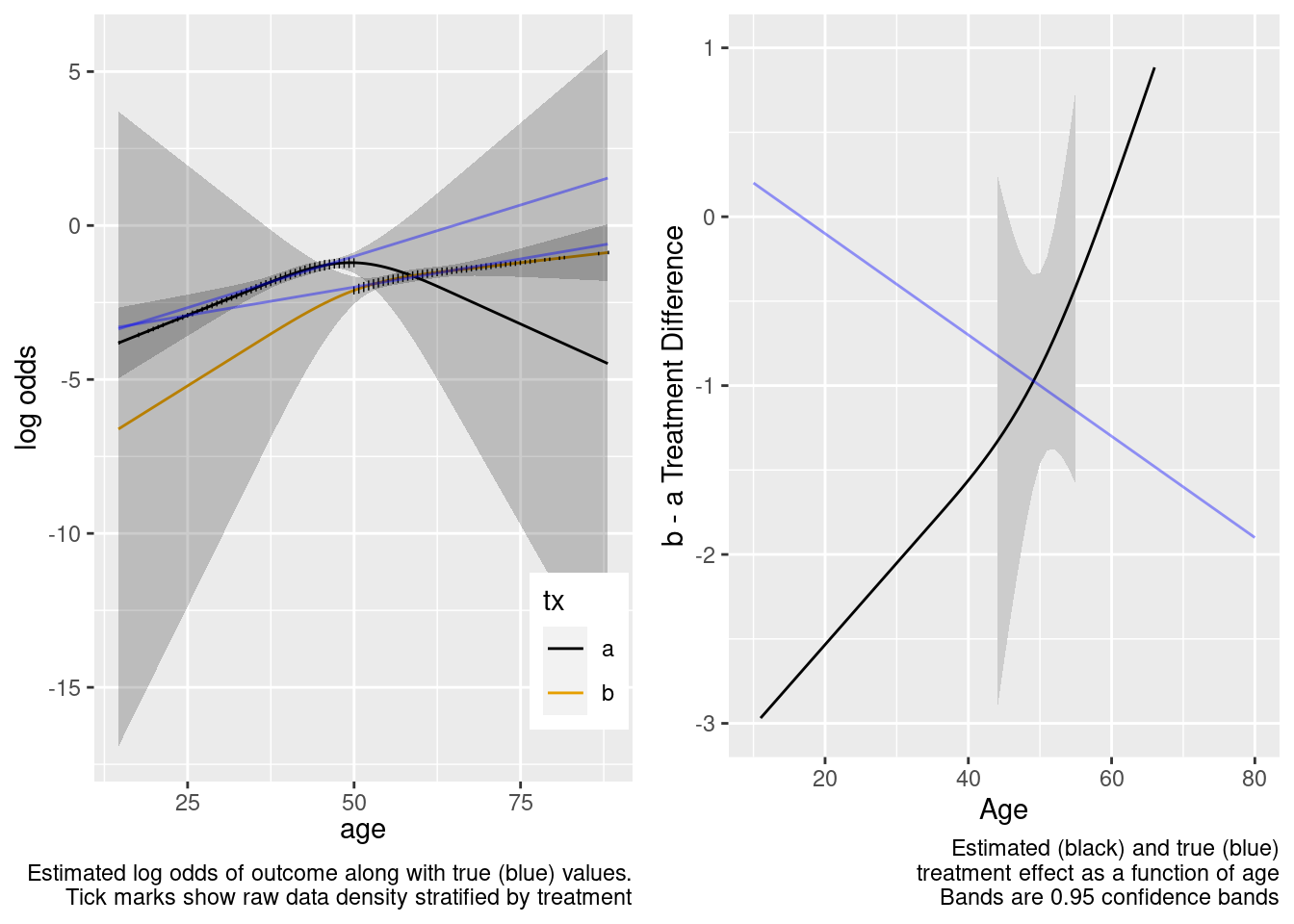Extrapolation failed, and the failure was thankfully signaled by very wide confidence bands for age-specific treatment effects.

This model correctly captures linear interaction, but also allows for unnecessary nonlinear interaction.

### Some Overlap in Ages Between Treatments

Logistic Regression Model

``` lrm(formula = y ~ tx + age, data = d)
```
Model Likelihood
Ratio Test
Discrimination
Indexes
Rank Discrim.
Indexes
Obs 3031 LR χ2 421.49 R2 0.189 C 0.732
0 2221 d.f. 2 R22,3031 0.129 Dxy 0.464
1 810 Pr(>χ2) <0.0001 R22,1780.6 0.210 γ 0.464
max |∂log L/∂β| 1×10-7 Brier 0.170 τa 0.182
β S.E. Wald Z Pr(>|Z|)
Intercept  -4.7976  0.3406 -14.09 <0.0001
tx=b  -0.7412  0.1640 -4.52 <0.0001
age   0.0751  0.0061 12.37 <0.0001
 χ2 d.f. P Wald Statistics for `y` tx 20.41 1 <0.0001 age 153.01 1 <0.0001 TOTAL 319.21 2 <0.0001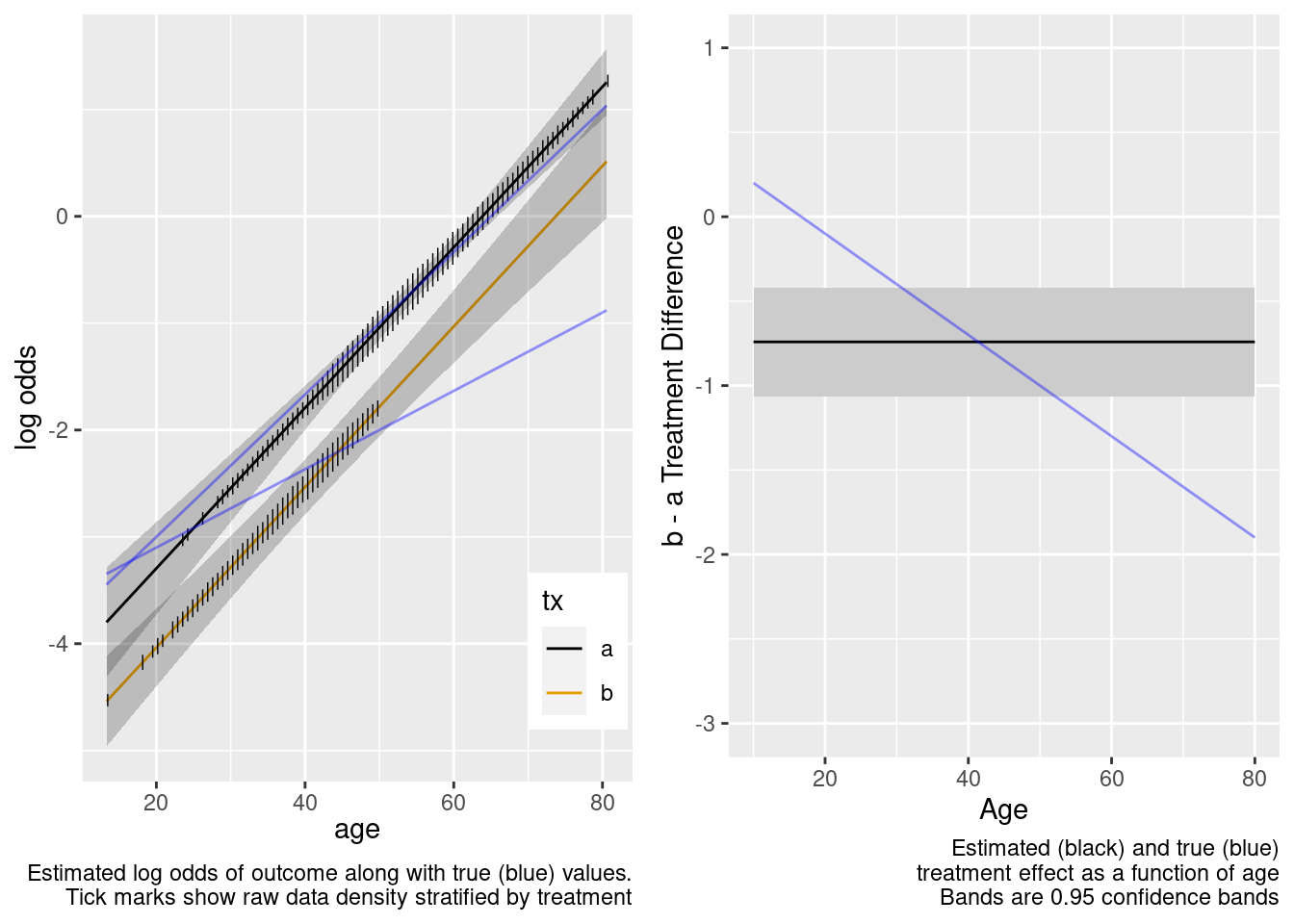The no-interaction model missed the boat. Now fit the correct linear interaction model.

This model failed to include a needed interaction term.

Logistic Regression Model

``` lrm(formula = y ~ tx * age, data = d)
```
Model Likelihood
Ratio Test
Discrimination
Indexes
Rank Discrim.
Indexes
Obs 3031 LR χ2 422.14 R2 0.189 C 0.732
0 2221 d.f. 3 R23,3031 0.129 Dxy 0.464
1 810 Pr(>χ2) <0.0001 R23,1780.6 0.210 γ 0.464
max |∂log L/∂β| 1×10-5 Brier 0.170 τa 0.182
β S.E. Wald Z Pr(>|Z|)
Intercept  -4.8638  0.3512 -13.85 <0.0001
tx=b   0.1333  1.0813 0.12 0.9019
age   0.0763  0.0063 12.18 <0.0001
tx=b × age  -0.0208  0.0255 -0.81 0.4151
 χ2 d.f. P Wald Statistics for `y` tx (Factor+Higher Order Factors) 20.56 2 <0.0001 All Interactions 0.66 1 0.4151 age (Factor+Higher Order Factors) 153.46 2 <0.0001 All Interactions 0.66 1 0.4151 tx × age (Factor+Higher Order Factors) 0.66 1 0.4151 TOTAL 326.02 3 <0.0001There is reasonable extrapolation. Now for the overkill model.

This is the correct model.

Logistic Regression Model

``` lrm(formula = form, data = d, tol = 1e-12)
```
Model Likelihood
Ratio Test
Discrimination
Indexes
Rank Discrim.
Indexes
Obs 3031 LR χ2 423.48 R2 0.190 C 0.732
0 2221 d.f. 5 R25,3031 0.129 Dxy 0.464
1 810 Pr(>χ2) <0.0001 R25,1780.6 0.209 γ 0.464
max |∂log L/∂β| 3×10-5 Brier 0.170 τa 0.182
β S.E. Wald Z Pr(>|Z|)
Intercept  -6.2165  1.3037 -4.77 <0.0001
tx=b   1.8369  1.9527 0.94 0.3468
age   0.1058  0.0280 3.78 0.0002
age’  -0.0262  0.0240 -1.09 0.2761
tx=b × age  -0.0602  0.0477 -1.26 0.2067
tx=b × age’   0.1114  0.2592 0.43 0.6672
 χ2 d.f. P Wald Statistics for `y` tx (Factor+Higher Order Factors) 16.32 3 0.0010 All Interactions 1.94 2 0.3784 age (Factor+Higher Order Factors) 151.01 4 <0.0001 All Interactions 1.94 2 0.3784 Nonlinear (Factor+Higher Order Factors) 1.30 2 0.5233 tx × age (Factor+Higher Order Factors) 1.94 2 0.3784 Nonlinear 0.18 1 0.6672 Nonlinear Interaction : f(A,B) vs. AB 0.18 1 0.6672 TOTAL NONLINEAR 1.30 2 0.5233 TOTAL NONLINEAR + INTERACTION 1.98 3 0.5774 TOTAL 325.31 5 <0.0001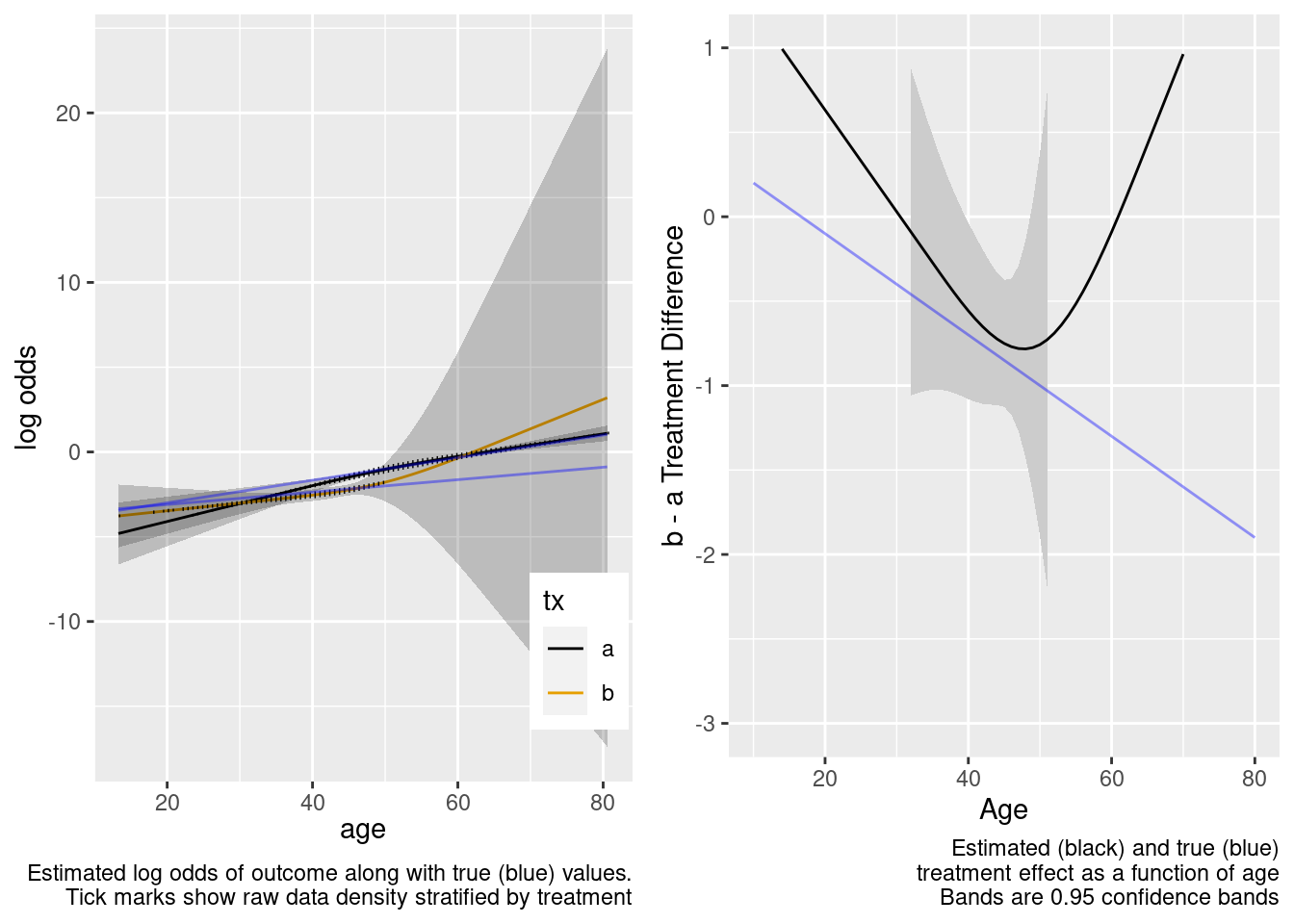Extrapolated treatment effect estimates are so-so.

This model correctly captures linear interaction, but also allows for unnecessary nonlinear interaction.

# Summary

With respect to relative efficacy (or both relative and absolute efficacy for continuous repsonse variables), clinical trials do not require having a representative sample of the target clinical population if there are no interactions with treatment. If there are interactions, let M denote the levels of interacting factors that are well represented in the target population. In our examples, M represents younger patients. Even with interaction present, the randomized trial still does not need to be on a representative patient sample if either (1) the trial sample is representative with respect to M (in which case omitting interactions from the model is not fatal), or (2) there is just enough representation in the sample with respect to M, and those interacting factors are appropriately modeled in the randomized trial. Unless M is richly represented in the trial, using statistical testing to decide on which interactions to include in the model is not advised, due to low power of such interaction tests. Suspected interactions, even statistically weak ones, should be included in the model when some degree of extrapolation is sought. When M is very poorly represented in the trial, extrapolation to the target population makes strong model assumptions. Thankfully confidence intervals for extrapolated efficacy estimates will be properly wide to reflect the weak basis for such extrapolation.

In a similar vein, observational treatment comparisons can be appropriate if factors that do not overlap between treatment groups do not interact with treatment. So a key to understanding both overlap and clinical trial generalizability is interactions.